# TypeScript学习第一章:TypeScript初识

## 1.1 TypeScript学习初见

TypeScript(TS)是由微软Microsoft由2012年推出的自由和开源的编程语言, 目前主流的三大框架React 、Vue 和 Angular这三大主流框架再加上最新的鸿蒙3.0都可以用TS进行开发.

## 1.2 TypeScript介绍

TS添加了可选的静态类型基于类的面向对象编程等等, 是JS的语言扩展, 不是JS的替代品, 会让JS前进的步伐更坚实、更遥远.

## 1.3 JS 、TS 和 ES之间的关系ES6又称为ECMAScript 2015, TypeScript 是 JS 的超集, 他包含Javascript的所有元素, 能运行Javascript代码, 并扩展了JS语法, 并添加了静态类型 模块 接口 类型注解等等方面的功能, 更加易于大项目的开发.

## 1.4 TS的竞争者有哪些?

### 1. ESLint### 2. TSlint1 和 2 都是和TypeScript一样来突出代码中可能出现的错误, 至少i没有为检查过程添加新的语法, 但是这两者都不打算最为IDE集成的工具来运行, 这两个的存在可以是TS做更少的检查, 但是这些检查并不适合于所有的代码库。

### 3. CoffeeScriptCoffeeScript是想改进JS语言, 但是现在用的人少了, 因为他又成为了JS的标准, 属于是打不过JS了。

### 4.Flow

Vue2的源码的类型检查工具就是flow, 不过Vue3已经开始使用TS做类型检查了.

Flow更悲观的判断类型, 而TS更加乐观.

Flow是为了维护Facebook的代码库而建立的, 而TS是作为一种独立的语言而建立的, 其内部有独立的环境, 可以自由专注于工具的开发整个生态系统的维护

# TypeScript学习第二章:为什么使用TypeScript?

## 2.1 发现问题

JS中每个值都有一组行为, 我们可以通过运行不同的操作来观察:

``````// 在 'message' 上访问属性方法 'toLowerCase', 并调用它
message.toLowerCase();
// 调用 'message'
message();
``````

``````const message = 'Hello World'
message.toLowerCase(); // 输出hello world
message(); // TypeError: message is not a function
``````

``````function fn(x) {
return x.flip()
}
``````

## 2.2 静态类型检查

``````const message = 'hello'
message() // TypeError
``````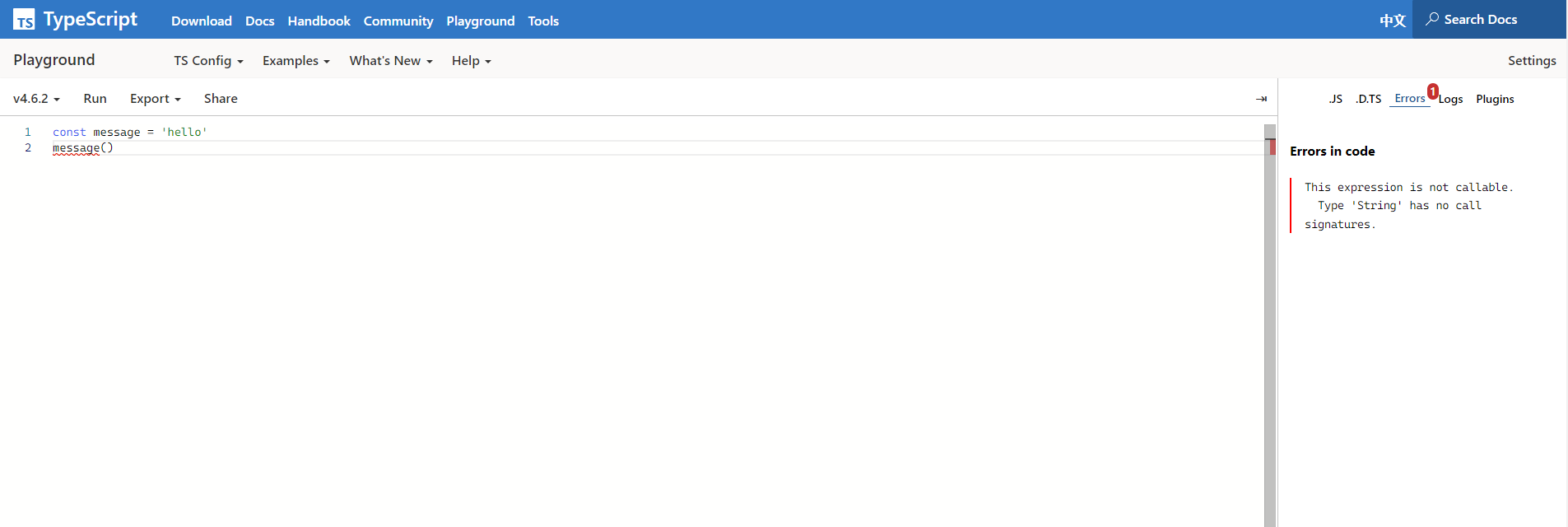## 2.3 非异常故障

JS 在运行的时候会告诉我们他认为某些东西是没有意义的情况, 因为ECMA规范明确说明了JS在遇到某些意外情况下应该是如何表现得, 比如如下代码:

``````const user = {
name: "小千",
age:26,
};

user.location; // 返回undefined, 理应报错, 因为根本没有location这个属性
``````TS可以在开发过程中捕获很多类似于合法的错误, 比如说错别字, 未调用函数, 基本的逻辑错误等等: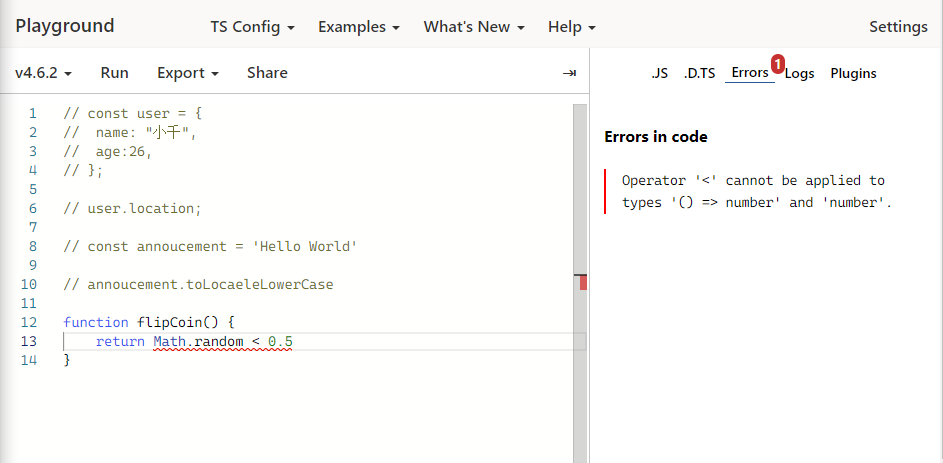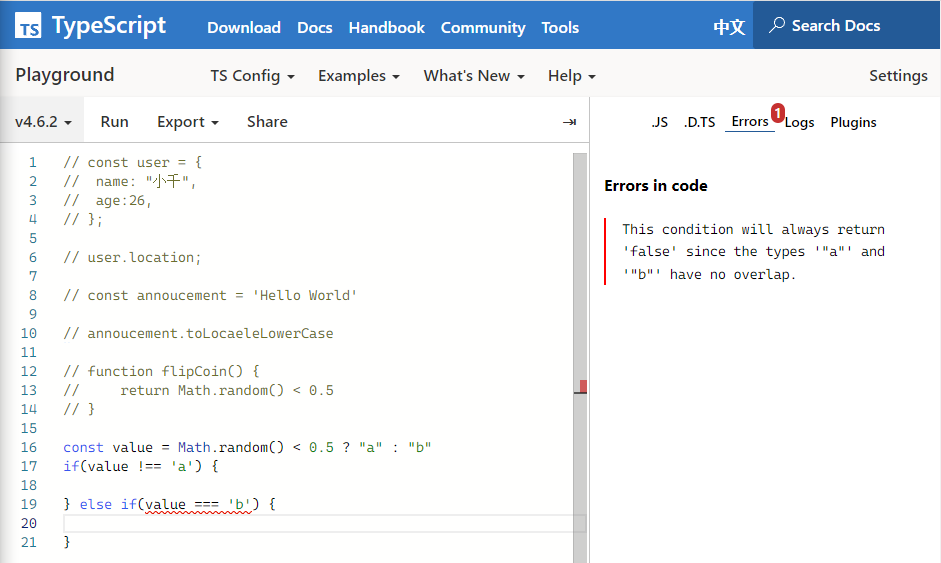## 2.4 使用工具

1. 安装VSCode
2. 安装Node.js:使用命令 `node -v`来检查nodejs版本
3. 安装TypeScript编译器: `npm i typescript -g`

hello.ts:

``````// 你好, 世界
// console.log('Hello World')

// 会出现函数实现重复的错误
function greet(person, date) {
console.log(`Helo \${person}, today is \${date}`)
}

greet('xiaoqian','2021/12/04')

``````

## 2.5 优化编译

1. 解决TS和JS冲突问题 `tsc --init # 生成配置文件`
2. 自动编译 `tsc --watch`
3. 发出错误 `tsc --noEmitOnError hello.ts`

TS文件编译成JS文件以后, 当出现函数名或者是变量名相同的时候, 会给我们提示重复定义的问题,可以通过` tsc --init`来生成一个配置文件来解决冲突问题. 先把严格模式strict关闭, 可解决未指定变量类型的问题.

``````tsc --watch --noEmitOnError
``````

## 2.6 显式类型

``````function greet(person: string, date: Date) {
console.log(`Helo \${person}, today is \${date.toDateString()}.`)
}
``````

## 2.7 降级编译

``````{
"compilerOptions": {
......
"target": 'es5',
......
}
}
``````

## 2.8 严格模式

``````{
"compilerOptions": {
......,
"strict": true, /* 严格模式: 启用所有严格的类型检查选项。*/
"noImplicitAny": true, /* 为隐含的'any'类型的表达式和声明启用错误报告。*/
"strictNullChecks": true, /* 当类型检查时，要考虑'null'和'undefined' */
......
}
}
``````

# TypeScript学习第三章: 常用类型

## 3.1 基元类型string number 和 boolean

1. string: 字符串, 例子: ‘Hello’, ‘World’.
2. number: 数字, 例子: 42, -100.
3. boolean: 布尔, 例子: true, false.

String Number Boolean 也是合法的, 在TS里专门指一些很少的, 出现在代码里的一些特殊的内置类型, 对于类型我们始终使用小写的string, number 和 boolean.

``````let str: string = 'hello typescript'
let num: number = 100
let bool: boolean = true
``````

## 3.2 数组

1. type[]
2. Array

Array

``````let arr: number[] = [1, 4, 6 ,8]
// arr = ['a']
let arr2: Array<number> = [1, 2, 3]
arr2 = []
``````

## 3.3 any

``````let obj: any = {
x: 0
}

obj.foo() // js调用时就会报错
obj()
obj.bar = 100
obj = 'hello'
const n: number = obj
``````

## 3.4 变量上的类型解释

``````let myName: string = "Felixlu"
``````

``````let my: string = "Hello World"
// 如果不声明, 会自动推断
let myName = "Bleak" // 将myName推断成string
myName = 100 // 报错, 不能将number分配给string.
``````

## 3.5 函数

``````function greet (name: string): void {
console.log("Hello," + name.toUpperCase() + "!!!")
}

const greet2 = (name: string): string =>{
return "你好," + name
}

greet("Bleak")
console.log(greet2("黯淡"))
``````

``````const names = ["xiaoqian", 'xiaoha', 'xiaoxi']
names.forEach(function(s) {
console.log(s.toUpperCase());
})

names.forEach(s => {
console.log(s.toLowerCase());
})
``````

## 3.6 对象类型

``````function printCoord(pt: {x: number; y: number}) {
console.log("坐标的x值是: " + pt.x)
console.log("坐标的y值是: " + pt.y)
}

printCoord({x: 3, y: 7})
``````

``````function printName(obj: {first: string, last?: string}) {
if(obj.last === undefined) {
console.log("名字是:" + obj.first)
} else {
console.log("名字是:" + obj.first + obj.last)
}

}

printName({
first: "Mr.",
last: "Bleak"
})
``````

1. ``````if(obj.last === undefined) {// 未传入时的方法体

} else {// 传入时的方法体

}
``````
2. ``````console.log(obj.last?.toUpperCase())
``````

## 3.7 联合类型

``````let id: number | string
``````

TS的类型系统允许我们使用多种运算符, 从现有类型中构建新类型union.

``````function printId(id: number | string) {
console.log("当前Id为:" + id)
// console.log(id.toUpperCase())
if (typeof id === 'string') {
console.log(id.toUpperCase())
} else {
console.log(id)
}
}

printId(101)
printId('202')
``````

``````function welcomePeople(x: string[] | string) {
if(Array.isArray(x)) { // Array.isArray(x)可以测试x是否是一个数组
console.log("Hello, " + x.join(' and '))
} else {
console.log("Welcome lone traveler " + x)
}
}

welcomePeople(["A", "B"])
welcomePeople('A')
``````

``````// 共享的方法
function getFirstThree(x: number[] | string) {
return x.slice(0, 3)
}
``````

## 3.8 类型别名

``````type Point = {
x: number
y: number
} // 对象类型
function printCoord(pt: Point) {

}
printCoord({x: 100, y: 200})

type ID = number | string // 联合类型
function printId(id: ID) {

}

printId(100)
printId('2333')

type UserInputSanitizedString = string // 基元类型
function sanitizedString(str: string): UserInputSanitizedString {
return str.slice(0, 2)
}

let userInput = sanitizedString('hello')
console.log(userInput)
``````

type可以用来定义变量的类型, 如果是对象, 里面的属性和方法可以用逗号, 分号; 或直接不写来做间隔, 可以用来做一些平时经常会用到的类型来做复用, 其可以用于变量的类型指定上.

## 3.9 接口

``````interface Point {
x: number;
y: number;
}

function printCoord(pt: Point) {
console.log("坐标x的值是: " + pt.x)
console.log("坐标y的值是: " + pt.y);
}
printCoord({ x: 100, y: 100 })

``````

1. 扩展接口: 通过extends
``````// 扩展接口
interface Animal {
name: string
}

interface Bear extends Animal {
honey: boolean
}

const bear: Bear = {
name: 'winie',
honey: true
}

console.log(bear.name, bear.honey)
``````

​ 扩展类型别名: 通过 &

``````type Animal  = {
name: string
}

type Bear = Animal & {
honey: boolean
}

const bear: Bear = {
name: "winie",
honey: true
}
``````
1. 向现有的类型添加新字段

接口: 定义相同的接口, 其字段会合并.

``````interface MyWindow {
count: number
}

interface MyWindow {
title: string
}

const w: MyWindow = {
title: 'hello ts',
count: 10
}
``````

​ 类型别名: 类型别名创建的类型创建后是不能添加新字段的

## 3.10 类型断言 as

``````const myCanvas = document.getElementById("main_canvas")  // 返回某种类型的HTMLElement

// 可以使用类型断言来指定
const myCanvas = document.getElementById("main_canvas") as HTMLCanvasElement
const myCanvas = <HTMLCanvasElement>document.getElementById()
``````

``````const x = ('hello' as unknown) as number
``````

## 3.11 文字类型

``````let testString = "Hello World";
testString = "Olá Mundo";

// 'testString'可以表示任何可能的字符串，那TypeScript是如何在类型系统中描述它的
testString;
const constantString = "Hello World";
// 因为'constantString'只能表示1个可能的字符串，所以具有文本类型表示
constantString;
``````

``````let x: "hello"  = "hello";
// 正确
x = "hello"
// 错误
x = "howdy"
``````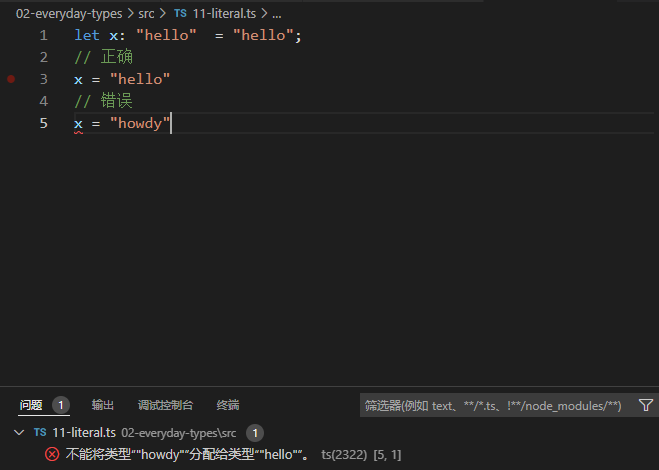``````function printText(s: string, alignment: "left" | "right" | "center") {
// ...
}
printText("Hello, world", "left");
printText("G'day, mate", "centre");
``````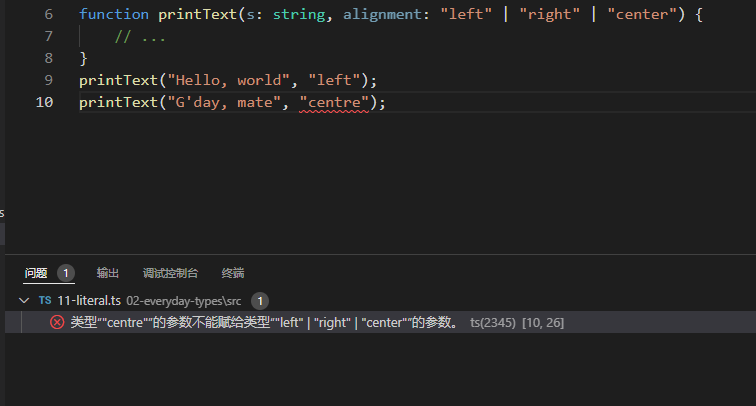``````function compare(a: string, b: string): -1 | 0 | 1 {
return a === b ? 0 : a > b ? 1 : -1;
}
``````

``````interface Options {
width: number;
}
function configure(x: Options | "auto") {
// ...
}
configure({ width: 100 });
configure("auto");
configure("automatic");
``````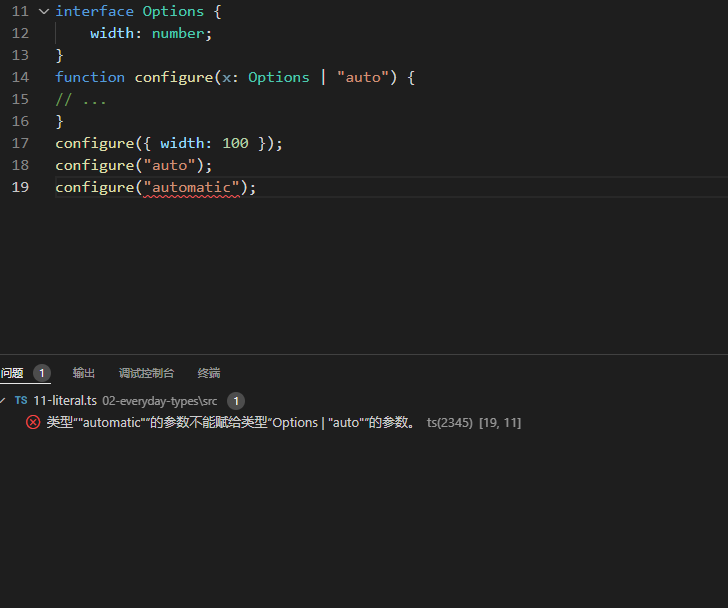### 文字推理

``````const obj = { counter: 0};
if(someCondtion) {
obj.counter = 1
}
``````

TypeScript 不假定先前具有的字段值 `0` ，后又分配 `1` 是错误的。另一种说法是 `obj.counter` 必须有 `number` 属性， 而非是 `0` ，因为类型用于确定读取和写入行为。

``````function handleRequest(url: string, method: 'GET' | 'POST' | 'GUESS') {
// ...
}
const req = { url: 'https://example.com', method: 'GET' };
handleRequest(req.url, req.method);
``````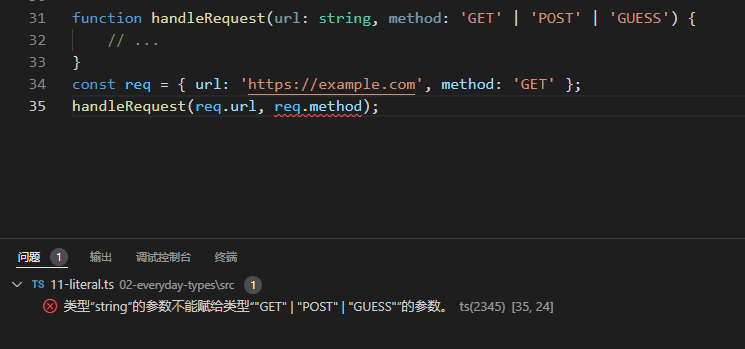1. 可以通过在任一位置添加类型断言来更改推理:
``````// 方案 1:
const req = { url: "https://example.com", method: "GET" as "GET" };
// 方案 2
handleRequest(req.url, req.method as "GET");
``````

1. 可以使用 `as const` 将整个对象转换为类型文字
``````const req = { url: "https://example.com", method: "GET" } as const;
handleRequest(req.url, req.method);
``````

`as const`后缀就像`const`定义，确保所有属性分配的文本类型，而不是一个更一般的`string``number`

## 3.12 `null`和`undefined`

JavaScript 有两个原始值用于表示不存在或未初始化的值： `null``undefined`.

TypeScript有两个对应的同名类型。这些类型的行为取决于您是否在`tsconfig.json`设置`strictNullChecks`选择。

• `strictNullChecks`关闭

使用false，仍然可以正常访问的值，并且可以将值分配给任何类型的属性。这类似于没有空检查的语言 （例如 C#、Java）的行为方式。缺乏对这些值的检查往往是错误的主要来源；如果在他们的代码库中这样做可行，我们总是建议大家打开。

• `strictNullChecks`开启

使用true，你需要在对该值使用方法或属性之前测试这些值。就像在使用可选属性之前检查一样，我们可以使用缩小来检查可能的值：

``````function doSomething(x: string | null) {
if (x === null) {
// 做一些事
} else {
console.log("Hello, " + x.toUpperCase());
}
}
``````
• 非空断言运算符(`!`后缀)

TypeScript 也有一种特殊的语法 `null``undefined` , 可以在不进行任何显式检查的情况下，从类型中移除和移除类型。 `!` 在任何表达式之后写入实际上是一种类型断言，即该值不是 `null` or `undefined`

``````function liveDangerously(x?: number | null) {
// 正确
console.log(x!.toFixed());
}
``````

## 3.13 枚举

``````// ts源码
enum Direction {
Up = 1,
Down,
Left,
Right,
}
console.log(Direction.Up) // 1
``````
``````// 编译后的js代码
"use strict";
var Direction;
(function (Direction) {
Direction[Direction["Up"] = 1] = "Up";
Direction[Direction["Down"] = 2] = "Down";
Direction[Direction["Left"] = 3] = "Left";
Direction[Direction["Right"] = 4] = "Right";
})(Direction || (Direction = {}));
console.log(Direction.Up);
``````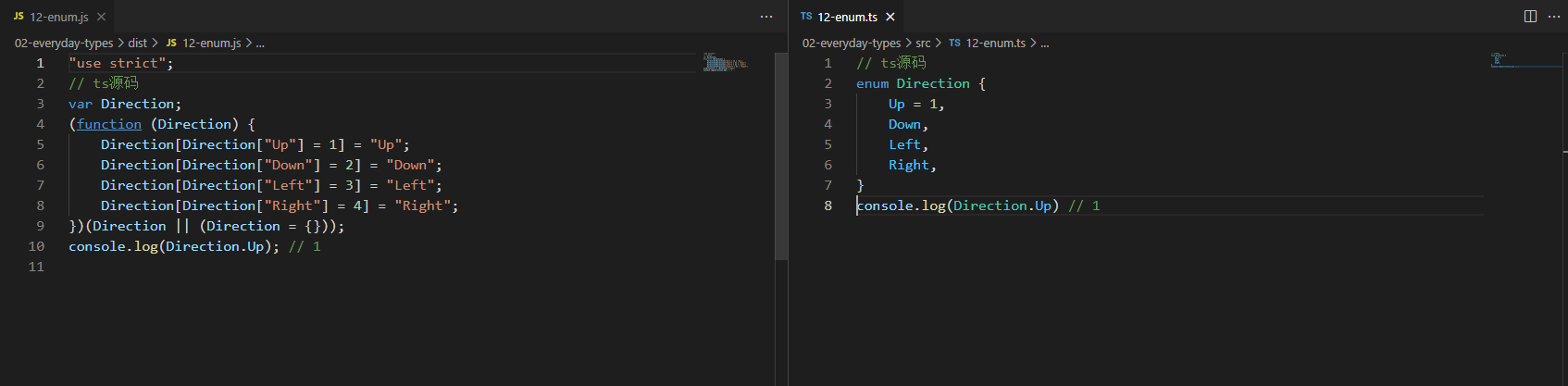## 3.14 不太常见的原语

• `bigint`

``````// 通过bigint函数创建bigint
const oneHundred: bigint = BigInt(100);
// 通过文本语法创建BigInt
const anotherHundred: bigint = 100n;
``````

• `symbol`

JavaScript 中有一个原语 `Symbol()` ，用于通过函数创建全局唯一引用：

``````const firstName = Symbol("name");
const secondName = Symbol("name");
if (firstName === secondName) {
// 这里的代码不可能执行
}
``````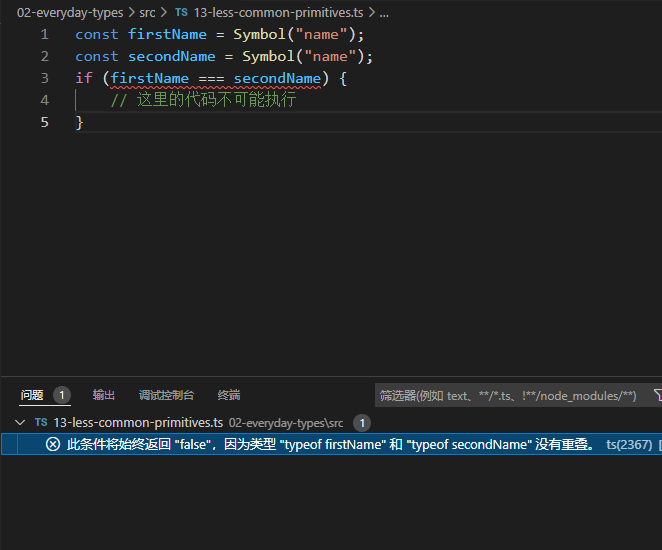# TypeScript学习第四章: 类型缩小

``````function padLeft(padding: number | string, input: string): string {
throw new Error("尚未实现!");
}
``````

``````function padLeft(padding: number | string, input: string): string {
return new Array(padding + 1).join(" ") + input;
}
``````

``````function padLeft(padding: number | string, input: string): string {
if (typeof padding === "number") {
return new Array(padding + 1).join(" ") + input;
}
return padding + input;

}
``````

TypeScript 可以理解几种不同的缩小结构.

## 4.1 `typeof`类型守卫

TS期望它返回一组特定的字符串:

• `"string"`
• `"number"`
• `"bigint"`
• `"boolean"`
• `"symbol"`
• `"undefined"`
• `"object"`
• `"function"`

``````function printAll(strs: string | string[] | null) {
if (typeof strs === "object") {
for (const s of strs) {
console.log(s);
}
} else if (typeof strs === "string") {
console.log(strs);
} else {
// 做点事
}
}
``````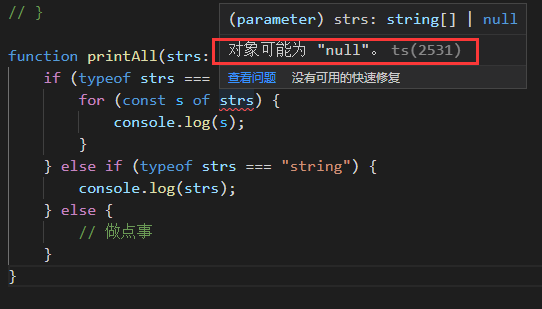`printAll` 函数中，我们尝试检查 `strs` 是否为对象，来代替检查它是否为数组类型（现在可能是强调数组是 JavaScript 中的对象类型的好时机）。但事实证明，在 JavaScript 中，` typeof null` 实际上也是 `"object"` ! 这是历史上的不幸事故之一。

## 4.2 真值缩小

``````function getUserOnlineMessage(numUserOnline: number) {
if(numUserOnline) {
return `现在共有 \${numUserOnline} 人在线!`
}
return "现在没有人在线:("
}
``````

• 0
• NaN
• “” (空字符串)
• On (bigint 0的版本)
• null
• undefined

``````// 这两个结果都返回 true
Boolean("hello"); // type: boolean, value: true
!!"world"; // type: true, value: true
``````

``````function printAll(strs: string | string[] | null) {
if (strs && typeof strs === "object") {
for (const s of strs) {
console.log(s);
}
} else if (typeof strs === "string") {
console.log(strs);
}
}
``````

``````TypeError: null is not iterable
``````

``````function printAll(strs: string | string[] | null) {
// !!!!!!!!!!!!!!!!
// 别这样!
// 原因在下边
// !!!!!!!!!!!!!!!!
if (strs) {
if (typeof strs === "object") {
for (const s of strs) {
console.log(s);
}
} else if (typeof strs === "string") {
console.log(strs);
}
}
}
``````

TS在这里根本不会报错, 如果你不熟悉JS, 这是值得注意的. TS通常可以帮你及早发现错误, 但是如果你选择对某个值不做任何处理, 那么它可以做的就只有这么多, 而不会考虑过多逻辑方面的问题, 如果需要, 你可以确保linter(程序规范性)处理此类情况.

``````function multiplyAll(
values: number[] | undefined,
factor: number
): number[] | undefined {
if (!values) {
return values;
} else {
return values.map((x) => x * factor);
}
}
``````

## 4.3 等值缩小

ts也使用分支语句做`===` `!==` `==``!=` 等值检查, 来实现类型缩小. 例如:

``````function example(x: string | number, y: string | boolean) {
if (x === y) {
// 现在可以在x,y上调用字符串类型的方法了
x.toUpperCase();
y.toLowerCase();
} else {
console.log(x);
console.log(y);
}
}
``````

``````function printAll(strs: string | string[] | null) {
if (strs !== null) {
if (typeof strs === "object") {
for (const s of strs) {
console.log(s);
}
} else if (typeof strs === "string") {
console.log(strs);
}
}
}
``````

JavaScript 更宽松的相等性检查 `==``!=` ，也能被正确缩小。如果你不熟悉，如何检查某个变量是否 `== null` ，因为有时不仅要检查它是否是特定的值 `null` ，还要检查它是否可能是 `undefined` 。这同样适用 于 `== undefined`它检查一个值是否为 null 或 undefined 。现在你只需要这个 `==``!=` 就可以搞定了。

``````interface Container {
value: number | null | undefined;
}
function multiplyValue(container: Container, factor: number) {
// 从类型中排除了undefined 和 null
if (container.value != null) {
console.log(container.value);
// 现在我们可以安全地乘以“container.value”了
container.value *= factor;
}
}

console.log(multiplyValue({value: 5}, 5))
console.log(multiplyValue({value: null}, 5))
console.log(multiplyValue({value: undefined}, 5))
console.log(multiplyValue({value: '5'}, 5))
``````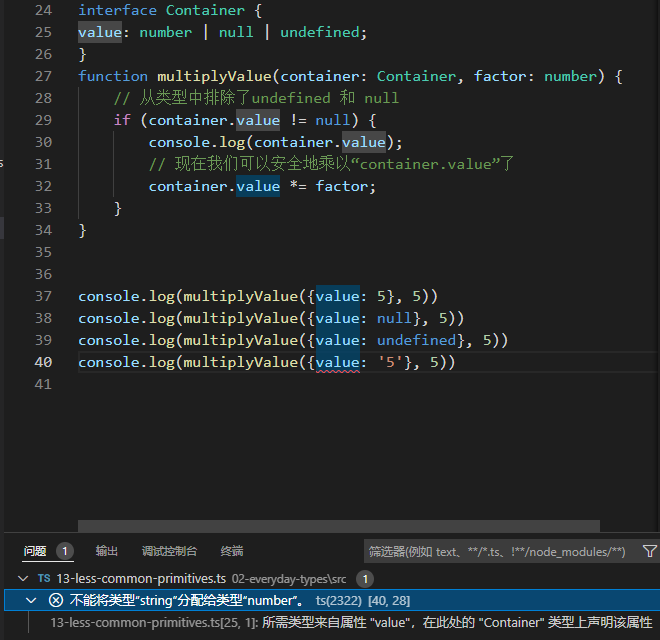## 4.4 `in`操作符缩小

JavaScript 有一个运算符，用于确定对象是否具有某个名称的属性： `in` 运算符。TypeScript 考虑到了这 一点，以此来缩小潜在类型的范围。 例如，使用代码： `"value" in x` 。这里的 `"value"`字符串string`x` 是联合类型。值为“true”的分支缩小，需要 x 具有可选或必需属性的类型的值；值为 `“false”` 的分支缩小，需要具有可选或缺失属性的类型的值

``````type Fish = { swim: () => void };
type Bird = { fly: () => void };
function move(animal: Fish | Bird) {
if ("swim" in animal) {
return animal.swim();
}
return animal.fly();
}
``````

``````type Fish = { swim: () => void };
type Bird = { fly: () => void };
type Human = { swim?: () => void; fly?: () => void };

function move(animal: Fish | Bird | Human) {
if ("swim" in animal) {
// animal: Fish | Human
animal;
} else {
// animal: Bird | Human
animal;
}
}
``````

## 4.5 `instanceof`操作符缩小

JS有一个运算符`instanceof`检查一个值是否是另一个值的“实例”。更具体地，在JavaScript 中 `x instanceof Foo` 检查 `x` 的原型链是否含有 `Foo.prototype` 。虽然我们不会在这里深入探讨，当 我们进入 `类(class)` 学习时，你会看到更多这样的内容，它们大多数可以使用 `new` 关键字实例化。 正如你可能已经猜到的那样， `instanceof` 也是一个类型保护，TypeScript 在由 `instanceof` 保护的分支中实现缩小。

``````function logValue(x: Date | string) {
if (x instanceof Date) {
console.log(x.toUTCString());
} else {
console.log(x.toUpperCase());
}
}
logValue(new Date()) // Mon, 15 Nov 2021 22:34:37 GMT
logValue('hello ts') // HELLO TS
``````

## 4.6 分配缩小

``````// let x: string | number
let x = Math.random() < 0.5 ? 10 : "hello world!";
x = 1;

// let x: number
console.log(x);
x = "goodbye!";
// let x: string
console.log(x);
``````

``````let x = Math.random() < 0.5 ? 10 : "hello world!";
// let x: string | number
x = 1;

// let x: number
console.log(x);

// 出错了~!
x = true

// let x: string | number
console.log(x);
``````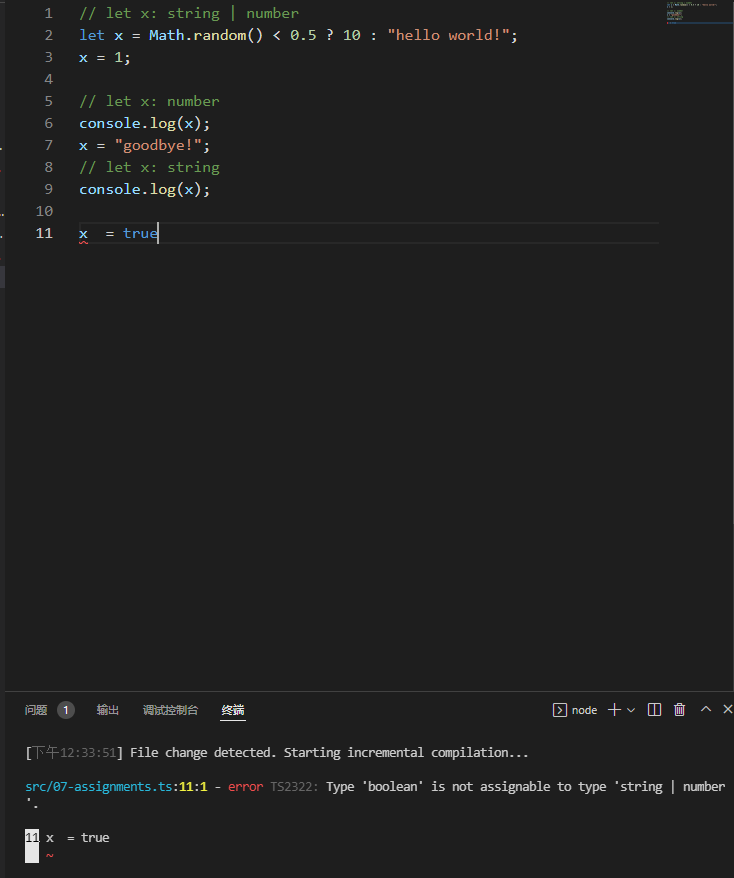## 4.7 控制流分析

``````function padLeft(padding: number | string, input: string) {
if (typeof padding === "number") {
return new Array(padding + 1).join(" ") + input;
}
return padding + input;
}
``````

padLeft从其第一个if块中返回. TS能够分析这段代码,并看到在padding是数字的情况下, 主体的其余部分（ `return padding + input;` ）是不可达的。因此，它能够将数字从 `padding` 的类型中移除（从string|number缩小到string），用于该函数的其余部分。

``````function example() {
let x: string | number | boolean;

x = Math.random() < 0.5;

// let x: boolean
console.log(x);
if (Math.random() < 0.5) {
x = "hello";
// let x: string
console.log(x);
} else {
x = 100;
// let x: number
console.log(x);
}
// let x: string | number
return x;
}
let x = example()
x = 'hello'
x = 100
x = true // error
``````## 4.8 使用类型谓词

``````type Fish = {
name: string
swim: () => void
}

type Bird = {
name: string
fly: () => void
}
function isFish(pet: Fish | Bird): pet is Fish {
return (pet as Fish).swim !== undefined
}
``````

``````function getSmallPet(): Fish | Bird {
let fish: Fish = {
name: 'gold fish',
swim: () => {
console.log('fish is swimming.')
}
}
let bird: Bird = {
name: 'sparrow',
fly: () => {
console.log('bird is flying.')
}
}
return Math.random() < 0.5 ? bird : fish
}
// 这里 pet 的 swim 和 fly 都可以访问了
let pet = getSmallPet() //
console.log(pet)
if (isFish(pet)) {
pet.swim()
} else {
pet.fly()
}
``````

``````const zoo: (Fish | Bird)[] = [getSmallPet(), getSmallPet(), getSmallPet()]
const underWater1: Fish[] = zoo.filter(isFish)
console.log(underWater1)
// 或者，等同于
const underWater2: Fish[] = zoo.filter(isFish) as Fish[]
console.log(underWater2)

// 对于更复杂的例子，该谓词可能需要重复使用
const underWatch3: Fish[] = zoo.filter((pet): pet is Fish => {
if (pet.name === 'frog') {
return false
}
return isFish(pet)
})
``````

## 4.9 受歧视的`unions`

``````interface Shape {
kind: "circle" | "square";
sideLength?: number;
}
``````

``````function handleShape(shape: Shape) {
// oops!
if (shape.kind === "rect") {
// ...
}
}
``````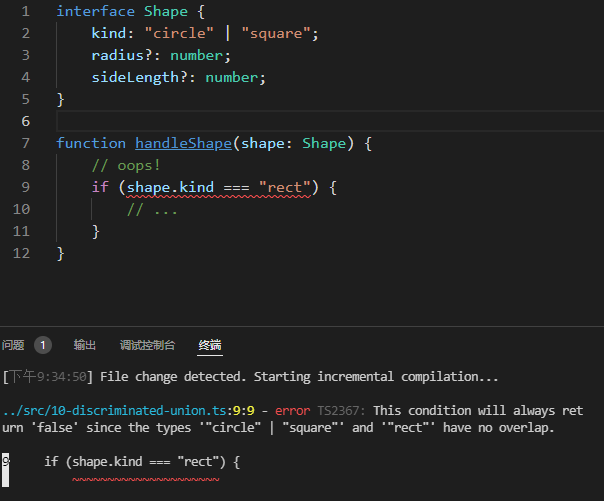``````function getArea(shape: Shape) {
return Math.PI * shape.radius ** 2;
}
``````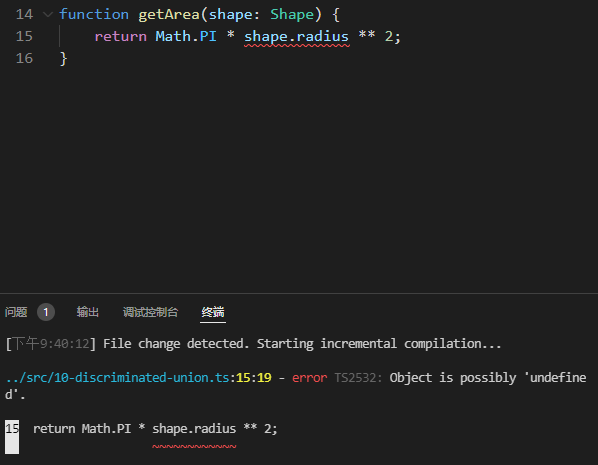`strictNullChecks`下，这给了我们一个错误——这是很恰当的，因为`radius`可能没有被定义。 但是如果我们对`kind`属性进行适当的检查呢？

``````function getArea(shape: Shape) {
if (shape.kind === "circle") {
return Math.PI * shape.radius ** 2;
}
}
``````

``````function getArea(shape: Shape) {
if (shape.kind === "circle") {
return Math.PI * shape.radius! ** 2;
}
}
``````

Shape 的这种编码的问题是，类型检查器没有办法根据种类属性知道 `radius``sideLength` 是否存在。我们需要把我们知道的东西传达给类型检查器。考虑到这一点，让我们再来定义一下`Shape`.

``````interface Circle {
kind: "circle";
}

interface Square {
kind: "square";
sideLength: number;
}
type Shape = Circle | Square;
``````

``````function getArea(shape: Shape) {
return Math.PI * shape.radius ** 2;
}
``````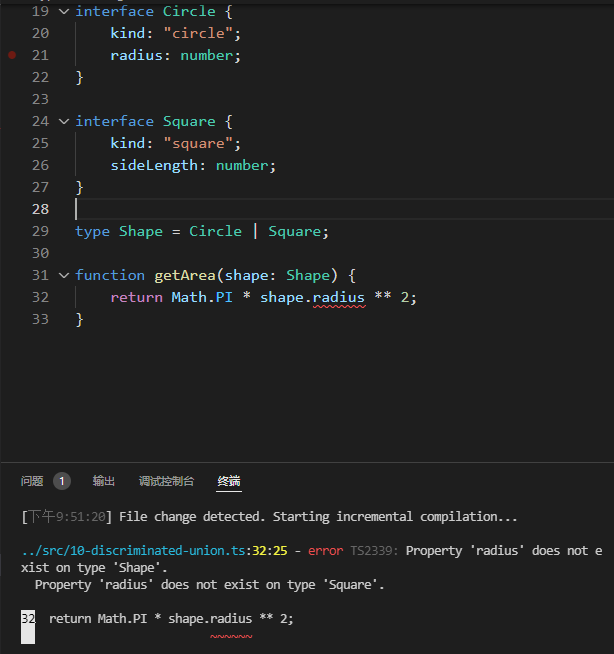``````function getArea(shape: Shape) {
if (shape.kind === "circle") {
// shape: Circle
return Math.PI * shape.radius ** 2;
}
}
``````

``````function getArea(shape: Shape) {
switch (shape.kind) {
// shape: Circle
case "circle":
return Math.PI * shape.radius ** 2;
// shape: Square
case "square":
return shape.sideLength ** 2;
}
}
``````

## 4.10 `never`类型与穷尽性检查

`never` 类型可以分配给每个类型；但是，没有任何类型可以分配给`never`（除了never本身）。这意味着你可以使用缩小并依靠 `never` 的出现在 `switch` 语句中做详尽的检查。

``````type Shape = Circle | Square;
function getArea(shape: Shape) {
switch (shape.kind) {
case "circle":
return Math.PI * shape.radius ** 2;
case "square":
return shape.sideLength ** 2;
default:
const _exhaustiveCheck: never = shape;
return _exhaustiveCheck;
}
}
``````

`Shape` 联盟中添加一个新成员，将导致TypeScript错误

``````interface Triangle {
kind: "triangle";
sideLength: number;
}
``````
``````type Shape = Circle | Square | Triangle;
function getArea(shape: Shape) {
switch (shape.kind) {
case "circle":
return Math.PI * shape.radius ** 2;
case "square":
return shape.sideLength ** 2;
default:
const _exhaustiveCheck: never = shape;
return _exhaustiveCheck;
}
}
``````# TypeScript学习第五章: 函数

## 5.1 函数类型表达式

``````function greeter(fn: (a: string) => void) {
fn("Hello, World");
}
function printToConsole(s: string) {
console.log(s);
}
greeter(printToConsole);
``````

``````type GreetFunction = (a: string) => void;
function greeter(fn: GreetFunction) {
// ...
}
``````

## 5.2 调用签名: 属性签名

``````type DescribableFunction = { // 对象类型
description: string // 函数的属性签名
(someArg: number): boolean // 函数类型表达式, 不能用=> 而是用:
}

function doSomething(fn: DescribableFunction) {
console.log(fn.description + " returned " + fn(6))
}

// 传入正常参数使用且正常返回值
function fn1(n: number) {
console.log(n)
return true
}
fn1.description = "hello"

// 不传入参数且不正常返回值
function fn2() {
console.log("lalala")
}
fn2.description = "heihei"

// 不传入参数且正常返回值
function fn3() {
return false
}
fn3.description = "hehehe"

doSomething(fn1) // 正常
doSomething(fn2) // 报错
doSomething(fn3) // 正常
``````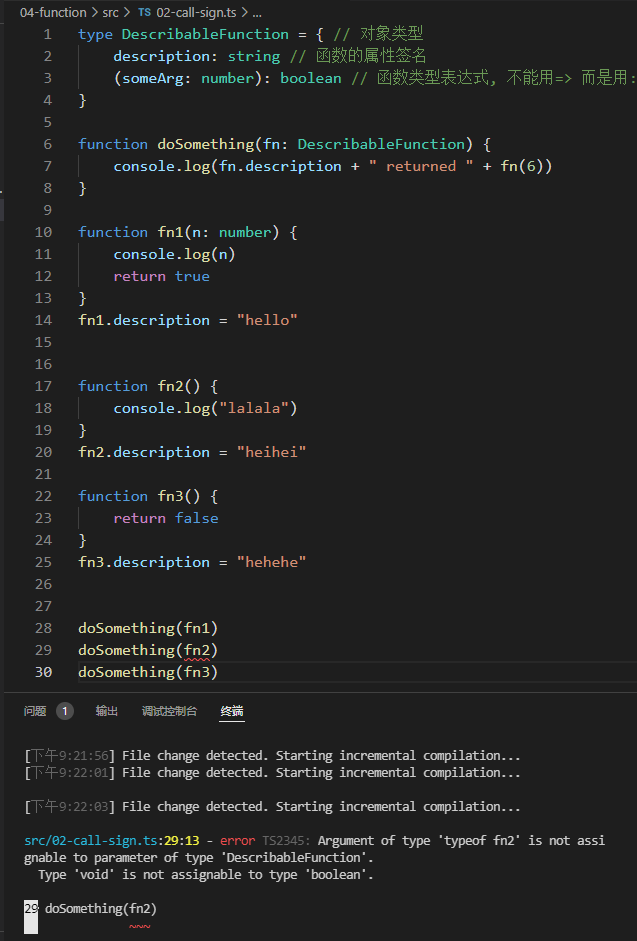## 5.3 构造签名 new (params, …): Ctor

JS函数也可以用`new`操作符来调用. TS将这些成为构造函数, 因为它们通常会创建一个新的对象。你可以通过在调用签名前面添加 `new` 关键字来写一个构造签名, 返回的是一个类或者构造函数.

``````class Ctor {
s: string
constructor(s: string) {
this.s = s
}
}

type SomeConstructor = { // 在调用签名前加new就是构造签名
new (s: string): Ctor // 返回的是一个构造函数或者类
}
function fn(ctor: SomeConstructor) { // SomeConstructor可以理解为构造函数
return new ctor("hello")
}

const f = fn(Ctor)
console.log(f.s)
``````

``````interface CallOrConstruct {
new (s: string): Date
(): string
}

function fn(date: CallOrConstruct) {
let d = new date('2021-11-20')
let n = date() // 因为Date可以在不使用new的情况下调用所以代码正常
console.log(d)
console.log(n)
}

fn(Date)
``````

``````// clock构造函数的接口, 是一个构造签名, 返回一个ClockInterface类的构造函数
interface ClockConstructor {
new (hour: number, minute: number): ClockInterface;
}

// Clock类的接口, 里面有一个tick()函数
interface ClockInterface {
tick(): void;
}

// 创建Clock类的函数
function createClock(
ctor: ClockConstructor,
hour: number,
minute: number
): ClockInterface {
return new ctor(hour, minute);
}

// 具体的类来实现ClockInterface, 必须要有tick函数
class DigitalClock implements ClockInterface {
h: number
m: number
constructor(h: number, m: number) {
this.h = h
this.m = m
}
tick() {
console.log("beep beep");
}
}

// 具体的类来实现ClockInterface, 必须要有tick函数
class AnalogClock implements ClockInterface {
h: number
m: number
constructor(h: number, m: number) {
this.h = h
this.m = m
}
tick() {
console.log("tick tock");
}
}

let digital = createClock(DigitalClock, 12, 17);
let analog = createClock(AnalogClock, 7, 32);
console.log(digital)
analog.tick()
``````

## 5.4 泛型函数`<Type>`

``````function firstElement(arr: any[]) {
return arr
}
``````

``````function firstElement<Type>(arr: Type[]): Type | undefined {
return arr
}
``````

``````// s 是 'string' 类型
const s = firstElement(["a", "b", "c"]);
// n 是 'number' 类型
const n = firstElement([1, 2, 3]);
// u 是 undefined 类型
const u = firstElement([]);
``````

### 5.4.1 类型推断

``````function map<Input, Output>(arr: Input[], func: (arg: Input) => Output): Output[] {
return arr.map(func)
}

// 参数'n'是'字符串'类型。
// 'parsed'是'number[]'类型。
const parsed = map(["1", "2", "3"], (n) => parseInt(n));
``````

### 5.4.2 限制条件

``````function longest<Type extends { length: number }>(a: Type, b: Type) {
if (a.length >= b.length) {
return a;
} else {
return b;
}
}
// longerArray 的类型是 'number[]'
const longerArray = longest([1, 2], [1, 2, 3]);
// longerString 是 'alice'|'bob' 的类型。
const longerString = longest("alice", "bob");
// 错误! 数字没有'长度'属性
const notOK = longest(10, 100);
``````

longerArray 和 longerString 的类型是根据参数推断出来的。记住，泛型就是把两个或多个具有相同类型的值联系起来。 最后，正如我们所希望的，对 longest(10, 100) 的调用被拒绝了，因为数字类型没有一个 `.length` 属性

### 5.4.3 使用受限值

``````function minimumLength<Type extends { length: number }>(
obj: Type,
minimum: number
): Type {
if (obj.length >= minimum) {
return obj
} else {
return { length: minimum }
}
}
``````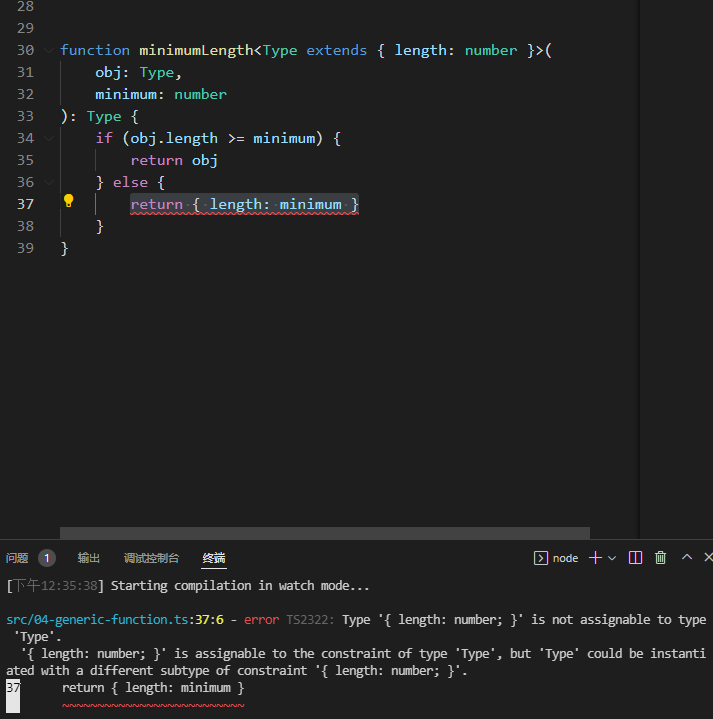``````// 'arr' 获得值： { length: 6 }
const arr = minimumLength([1, 2, 3], 6);
//在此崩溃，因为数组有一个'切片'方法，但没有返回对象!
console.log(arr.slice(0));
``````

### 5.4.4 指定类型参数

TypeScript 通常可以推断出通用调用中的预期类型参数，但并非总是如此。例如，假设你写了一个函数来合并两个数组：

``````function combine<Type>(arr1: Type[], arr2: Type[]): Type[] {
return arr1.concat(arr2)
}
``````

``````const arr = combine([1, 2, 3], ["hello"]);
``````

``````const arr = combine<string | number>([1, 2, 3], ["hello"])
``````

### 5.4.5 编写优秀通用函数的准则

• 类型参数下推

``````function firstElement1<Type>(arr: Type[]) {
return arr;
}
function firstElement2<Type extends any[]>(arr: Type) {
return arr;
}
// a: number (推荐)
const a = firstElement1([1, 2, 3]);
// b: any (不推荐)
const b = firstElement2([1, 2, 3]);
``````

• 使用更少的类型参数

``````function filter1<Type>(arr: Type[], func: (arg: Type) => boolean): Type[] {
return arr.filter(func);
}
function filter2<Type, Func extends (arg: Type) => boolean>(
arr: Type[],
func: Func
): Type[] {
return arr.filter(func);
}
``````

• 类型参数应该出现两次及以上

``````function greet<Str extends string>(s: Str) {
console.log("Hello, " + s);
}
greet("world");
``````

``````function greet(s: string) {
console.log("Hello, " + s);
}
``````

## 5.5 可选参数 ?

JavaScript中的函数经常需要一个可变数量的参数。例如，`number``toFixed` 方法需要一个可选的数字计数。

``````function f(n: number) {
console.log(n.toFixed()); // 0 个参数
console.log(n.toFixed(3)); // 1 个参数
}
``````

``````function f(x?: number) {
// ...
}
f(); // 正确
f(10); // 正确

``````

``````function f(x = 10) {
//...
}
``````

``````declare function f(x?: number): void;

// 以下调用都是正确的
f();
f(10);
f(undefined);
``````

### 5.5.1 回调中的可选参数

``````function myForEach(arr: any[], callback: (arg: any, index?: number) => void) {
for (let i = 0; i < arr.length; i++) {
callback(arr[i], i);
}
}
``````

``````myForEach([1, 2, 3], (a) => console.log(a))
myForEach([1, 2, 3], (a, i) => console.log(a, i))
``````

``````function myForEach(arr: any[], callback: (arg: any, index?: number) => void) {
for (let i = 0; i < arr.length; i++) {
// 我现在不想提供索引
callback(arr[i]);
}
}
``````

``````myForEach([1, 2, 3], (a, i) => {
console.log(i.toFixed())
})
``````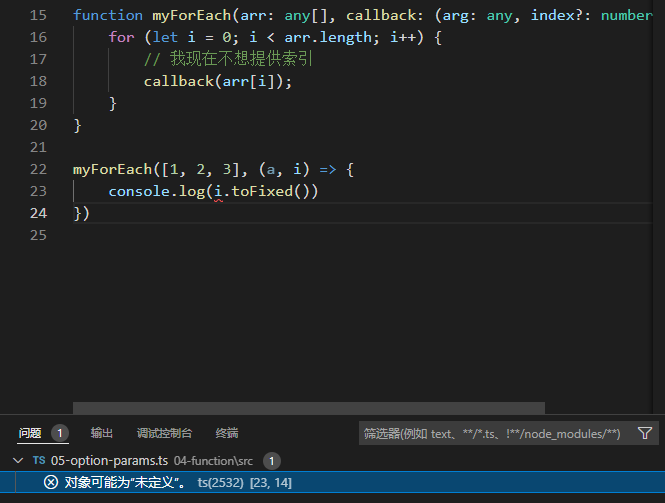## 5.6 函数重载: 重载签名

``````function makeDate(timestamp: number): Date // 重载签名
function makeDate(m: number, d: number, y: number): Date // 重载签名
function makeDate(mOrTimestamp: number, d?: number, y?: number): Date {
if(d !== undefined && y !== undefined) {
return new Date(y, mOrTimestamp, d)
} else {
return new Date(mOrTimestamp)
}
}

const d1 = makeDate(12345678)
const d2 = makeDate(5,5,5)
const d3 = makeDate(1, 3)
``````### 5.6.1 重载签名和实现签名

``````function fn(x: string): void
function fn() {
// ...
}
// 期望能够以零参调用
fn()
````````````function fn(x: boolean): void;
// 参数类型不正确
function fn(x: string): void;
function fn(x: boolean) {}
``````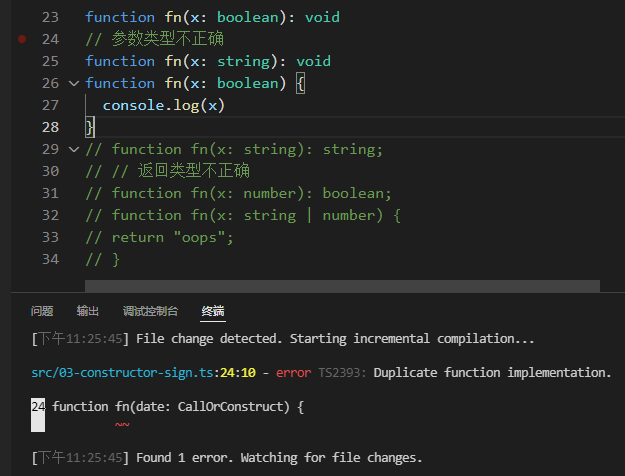``````function fn(x: string): string
// 返回类型不正确
function fn(x: number): boolean
function fn(x: string | number) {
return "oops";
}
``````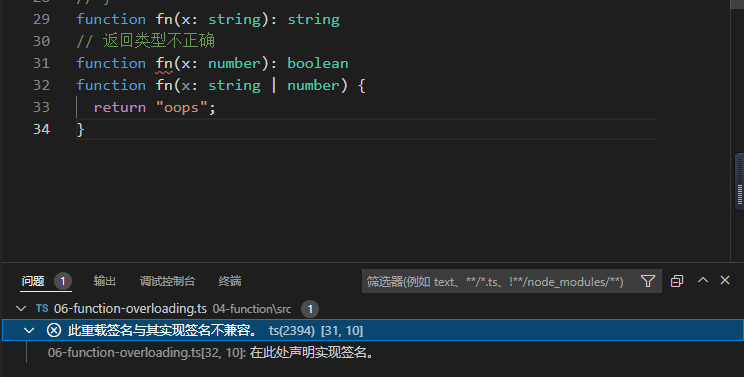### 5.6.2 编写好的重载

``````function len(s: string): number;
function len(arr: any[]): number;
function len(x: any) {
return x.length;
}
``````

``````len(""); // OK
len(); // OK
len(Math.random() > 0.5 ? "hello" : );
``````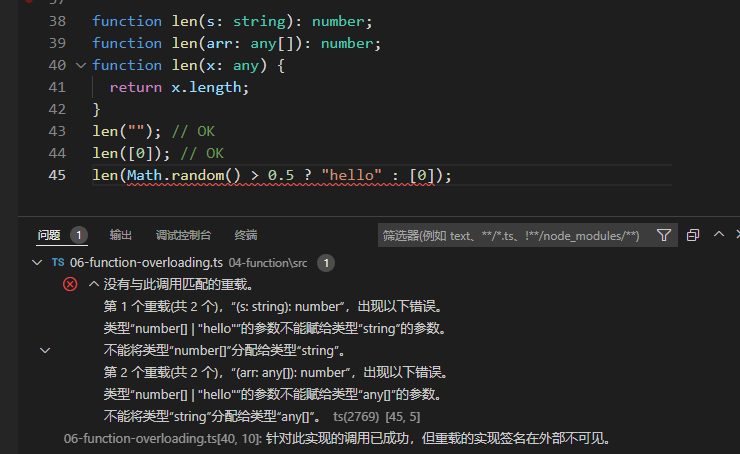``````function len(x: any[] | string) {
return x.length;
}
len(""); // OK
len(); // OK
len(Math.random() > 0.5 ? "hello" : ); // OK
``````

### 5.6.3 函数内`This`的声明

TS会通过代码分析来推断函数中`this`应该是什么, 比如下面的例子:

``````const user = {
id: 123,
becomeAdmin: function () {
},
};
``````

TS理解函数`user.becomeAdmin`有一个对应的`this`, 它是外部对象`user`. 这个对于很多情况来说已经足够了, 但是有很多情况下你需要更多的控制`this`代表什么对象/

JavaScript规范规定， 你不能有一个叫 `this` 的参数，所以TypeScript使用这个语法空间，让你在函数体中声明 `this` 的类型。

``````interface Card {
suit: string;
card: number;
}
interface Deck {
suits: string[];
cards: number[];
createCardPicker(this: Deck): () => Card;
}
let deck: Deck = {
suits: ["hearts", "spades", "clubs", "diamonds"],
cards: Array(52),
// NOTE: The function now explicitly specifies that its callee must be of type Deck
createCardPicker: function(this: Deck) {
return () => {
let pickedCard = Math.floor(Math.random() * 52);
let pickedSuit = Math.floor(pickedCard / 13);

return {suit: this.suits[pickedSuit], card: pickedCard % 13};
}
}
}

console.log(deck.createCardPicker()())
``````

## 5.7 需要了解的其他类型

### 5.7.1 `void`

`void`表示没有返回值的函数的返回值. 当一个函数没有任何返回语句, 或者没有从这些返回语句中返回任何明确的值时, 它都是推断出来`void`类型.

``````// 推断出的返回类型是void
function noop() {
return;
}
``````

void 和 undefined是不一样的

### 5.7.2 `object`

`object` 不是 `Object` 。始终使用 `object` !

### 5.7.3 `unknown`

`unknown`类型代表任何值. 这与`any`类型相似, 但更安全, 因为对未知`unknown`值做任何事情都是不合法的.

``````function f1(a: any) {
a.b(); // 正确
}
function f2(a: unknown) {
a.b();
}
``````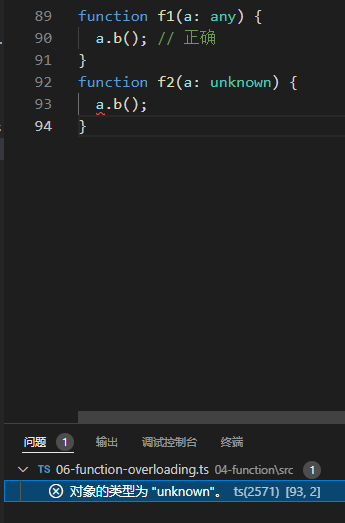``````function safeParse(s: string): unknown {
return JSON.parse(s);
}

// 需要小心对待'obj'!
const obj = safeParse(someRandomString);
``````

### 5.7.4 `never`

``````function fail(msg: string): never {
throw new Error(msg);
}
``````

`never` 类型标识永远不会被观察到的值. 载一个返回类型中, 这意味着函数抛出了一个异常或终止程序的执行.

never 也出现在TypeScript确定一个 `union` 中没有任何东西的时候。

``````function fn(x: string | number) {
if (typeof x === "string") {
// 做一些事
} else if (typeof x === "number") {
// 再做一些事
} else {
x; // 'never'!
}
``````

### 5.7.5 `Function`

``````function doSomething(f: Function) {
return f(1, 2, 3);
}
``````

## 5.8 函数展开运算符

### 5.8.1 形参展开(Rest Parameters)

`rest` 参数出现在所有其他参数之后，并使用 `...` 的语法：

``````function multiply(n: number, ...m: number[]) {
return m.map((x) => n * x);
}
// 'a' 获得的值 [10, 20, 30, 40]
const a = multiply(10, 1, 2, 3, 4);
``````

### 5.8.2 实参展开(Rest Arguments)

``````const arr1 = [1, 2, 3];
const arr2 = [4, 5, 6];
arr1.push(...arr2);
console.log(arr1) //[ 1, 2, 3, 4, 5, 6 ]
``````

``````// 推断的类型是 number[] -- "一个有零或多个数字的数组"。
// 不专指两个数字
const args = [8, 5];
const angle = Math.atan2(...args);
````````````// 推断为2个长度的元组
const args = [8, 5] as const;
// 正确
const angle = Math.atan2(...args);
``````

## 5.9 参数解构

``````function sum({ a, b, c }) {
console.log(a + b + c);
}
sum({ a: 10, b: 3, c: 9 });
``````

``````function sum( { a, b, c }: { a: number, b: number, c: number }) {
console.log(a + b + c)
}
``````

``````// 与之前的例子相同
type ABC = { a: number; b: number; c: number };
function sum({ a, b, c }: ABC) {
console.log(a + b + c);
}
``````

## 5.10 函数的可分配性: 返回`void`类型

``````type voidFunc = () => void
const f1: voidFunc = () => {
return true
}
const f2: voidFunc = () => true
const f3: voidFunc = function () {
return true
}
``````

``````const v1 = f1();
const v2 = f2();
const v3 = f3();
console.log(v1) // true
console.log(v2) // true
console.log(v3) // true
``````

``````const src = [1, 2, 3];
const dst = ;
src.forEach((el) => dst.push(el));
``````

``````function f2(): void {
return true;
}
const f3 = function (): void {
return true;
}
``````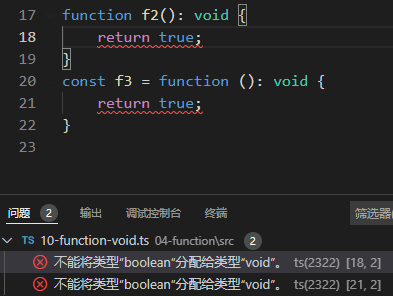# TypeScript学习第六章: 对象类型

``````function greet(person: { name: string; age: number }) { // 匿名对象{ name: string; age: number }
return "Hello " + person.name;
}
``````

``````interface Person { // 接口中定义了一个对象类型,包含name和age
name: string
age: number
}

function greet(person: Person) {
return 'Hello ' + person.name
}
``````

``````type Person = { // 类型别名种定义了一个对象类型, 其包含name和age
name: string;
age: number;
};
function greet(person: Person) {
return "Hello " + person.name;
}
``````

## 6.2 可选属性

``````type Shape = {}

interface PaintOptions {
shape: Shape;
xPos?: number;
yPos?: number;
}

function paintShape(opts: PaintOptions) {
// ...
}

const shape: Shape = {}
paintShape({ shape });
paintShape({ shape, xPos: 100 });
paintShape({ shape, yPos: 100 });
paintShape({ shape, xPos: 100, yPos: 100 });
``````

``````function paintShape(opts: PaintOptions) {
let xPos = opts.xPos;
let yPos = opts.yPos;
// ...
}
``````

``````function paintShape(opts: PaintOptions) {
let xPos = opts.xPos === undefined ? 0 : opts.xPos;
let yPos = opts.yPos === undefined ? 0 : opts.yPos;
// ...
}
``````

``````function paintShape({ shape, xPos = 0, yPos = 0 }: PaintOptions) {// 注意, 此时用了解构的语法, 将PaintOptions里的参数结构出来, 并给xPos和yPos设置了默认值
console.log("x coordinate at", xPos);
console.log("y coordinate at", yPos);
// ...
}
``````

``````function redner(args: Shape | number) {}
function draw({ shape: Shape, xPos: number = 100 /*...*/ }) {
render(shape);
render(xPos);
}
``````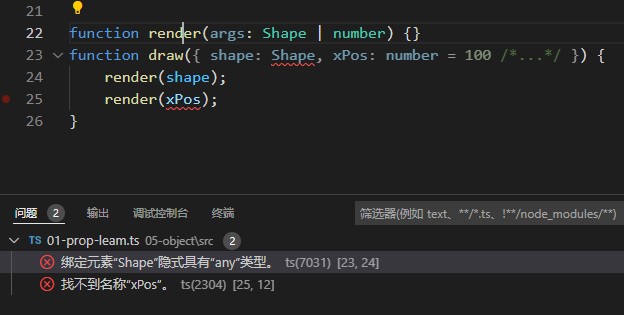## 6.3 只读属性

``````interface SomeType {
}
function doSomething(obj: SomeType) {
// 可以读取 'obj.prop'.
console.log(`prop has the value '\${obj.prop}'.`);
// 但不能重新设置值
obj.prop = "hello";
}
``````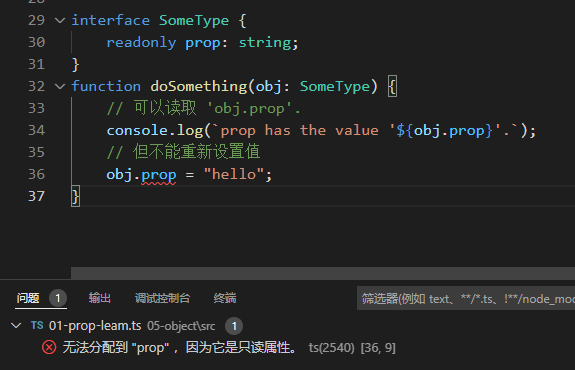``````interface Home {
readonly resident: { name: string; age: number };
}
function visitForBirthday(home: Home) {
// 我们可以从'home.resident'读取和更新属性。
console.log(`Happy birthday \${home.resident.name}!`);
home.resident.age++;
}
function evict(home: Home) {
// 但是我们不能写到'home'上的'resident'属性本身。
home.resident = {
name: "Victor the Evictor",
age: 42,
};
}
``````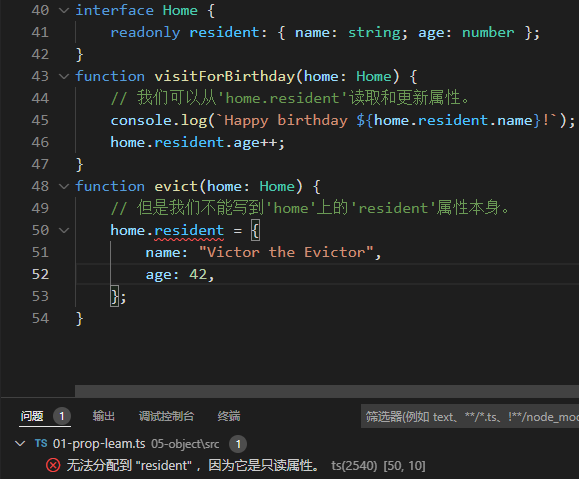``````interface Person {
name: string;
age: number;
}
}
let writablePerson: Person = {
name: "Person McPersonface",
age: 42,
};
// 正常工作
console.log(readonlyPerson.age); // 打印 '42'
writablePerson.age++;
console.log(readonlyPerson.age); // 打印 '43'
``````

## 6.4 索引签名

``````interface StringArray {
[index: number]: string;
}
const myArray: StringArray = ['a', 'b'];
const secondItem = myArray;
``````

``````interface Animal {
name: string;
}
interface Dog extends Animal {
breed: string;
}
interface NotOkay {
[x: number]: Animal;
[x: string]: Dog;
}
``````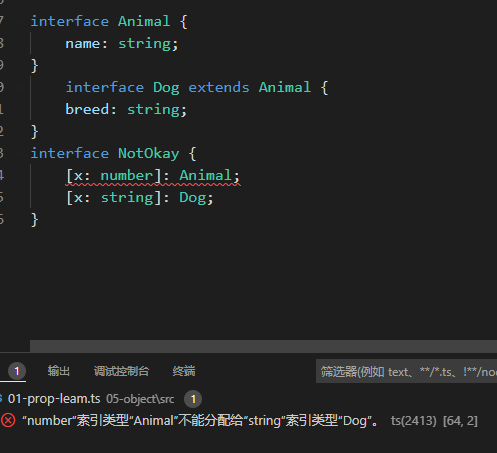``````interface NumberDictionary {
[index: string]: number;
length: number; // ok
name: string; // error
}
``````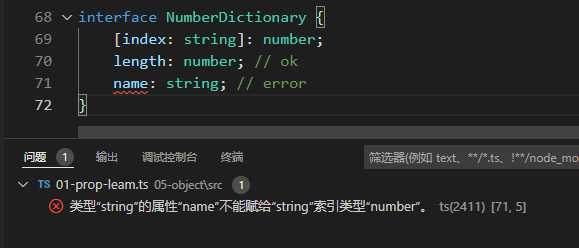``````interface NumberOrStringDictionary {
[index: string]: number | string;
length: number; // 正确, length 是 number 类型
name: string; // 正确, name 是 string 类型
}
``````

``````interface ReadonlyStringArray {
readonly [index: number]: string;
}
myArray = "Mallory";
``````

## 6.5 扩展类型

``````interface BasicAddress {
name?: string;
street: string;
city: string;
country: string;
postalCode: string;
}
``````

``````interface AddressWithUnit {
name?: string;
unit: string;
street: string;
city: string;
country: string;
postalCode: string;
}
``````

``````interface BasicAddress {
name?: string;
street: string;
city: string;
country: string;
postalCode: string;
}

unit: string;
}
``````

``````interface Colorful {
color: string;
}
interface Circle {
}
interface ColorfulCircle extends Colorful, Circle {}

const cc: ColorfulCircle = {
color: "red",
}
``````

## 6.6 交叉类型

``````interface Colorful {
color: string;
}
interface Circle {
}
type ColorfulCircle = Colorful & Circle;

const cc: ColorfulCircle = {
color: "red",
}
``````

``````function draw(circle: Colorful & Circle) {
console.log(`Color was \${circle.color}`);
}
// 正确
draw({ color: "blue", radius: 42 });
// 错误
draw({ color: "red", raidus: 42 });
``````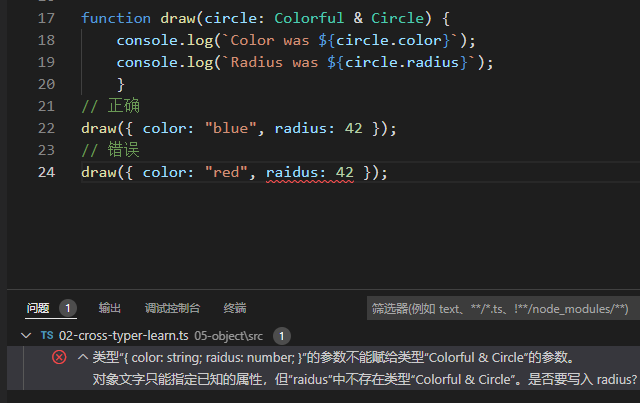## 6.7 接口与交叉类型

``````interface Sister {
name: string
}

interface Sister {
age: number
}
const sisterAn: Sister = {
name: "sisterAn"
}
const sisterRan: Sister = {
name: "sisterRan",
age: 12
}
````````````type Sister = {
name: string;
}
type Sister = {
age: number;
}
``````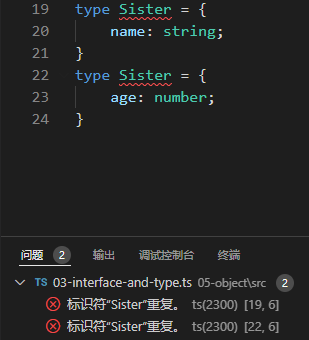## 6.8 泛型对象类型

``````interface Box {
contents: any;
}
``````

``````interface Box {
contents: unknown;
}

let x: Box = {
contents: "hello world",
};

// 我们需要检查 'x.contents'
if (typeof x.contents === "string") {
console.log(x.contents.toLowerCase());
}

// 或者用类型断言
console.log((x.contents as string).toLowerCase());
``````

``````interface NumberBox {
contents: number;
}

interface StringBox {
contents: string;
}

interface BooleanBox {
contents: boolean;
}
``````

``````function setContents(box: StringBox, newContents: string): void;
function setContents(box: NumberBox, newContents: number): void;
function setContents(box: BooleanBox, newContents: boolean): void;
function setContents(box: { contents: any }, newContents: any) {
box.contents = newContents;
}
``````

``````interface Box<Type> {
contents: Type
}
``````

``````let box: Box<string>
``````

`Box` 想象成一个真实类型的模板，其中 `Type` 是一个占位符，会被替换成其他类型。当 TypeScript看到 `Box<string> `时，它将用字符串替换 `Box<Type>` 中的每个 `Type` 实例，并最终以 `{ contents: string }` 这样的方式工作。换句话说， `Box` 和我们之前的 `StringBox` 工作起来是一样的。

``````interface Box<Type> {
contents: Type;
}
interface StringBox {
contents: string;
}
let boxA: Box<string> = { contents: "hello" };
boxA.contents;

let boxB: StringBox = { contents: "world" };
boxB.contents;

``````

``````interface Box<Type> {
contents: Type;
}

interface Apple {
// ....
}

// 等价于 '{ contents: Apple }'.
type AppleBox = Box<Apple>;
``````

``````function setContents<Type>(box: Box<Type>, newContents: Type) {
box.contents = newContents;
}
``````

``````type Box<Type> = {
contents: Type;
}
``````

``````type OrNull<Type> = Type | null;
type OneOrMany<Type> = Type | Type[];
type OneOrManyOrNull<Type> = OrNull<OneOrMany<Type>>;
type OneOrManyOrNullStrings = OneOrManyOrNull<string>;
``````

## 6.9 数组类型

``````function doSomething(value: Array<string>) {
// ...
}

let myArray: string[] = ["hello", "world"];

// 这两样都能用
doSomething(myArray);
doSomething(new Array("hello", "world"));
``````

``````interface Array<Type> {
/**
* 获取或设置数组的长度。
*/
length: number;

/**
* 移除数组中的最后一个元素并返回。
*/
pop(): Type | undefined;

/**
* 向一个数组添加新元素，并返回数组的新长度。
*/
push(...items: Type[]): number;
// ...
}
``````

## 6.10 只读数组类型

`ReadonlyArray` 是一个特殊的类型，描述了不应该被改变的数组。

``````function doStuff(values: ReadonlyArray<string>) {
// 我们可以从 'values' 读数据...
const copy = values.slice();
console.log(`第一个值是 \${values}`);
// ...但我们不能改变 'vulues' 的值。
values.push("hello!");
}
``````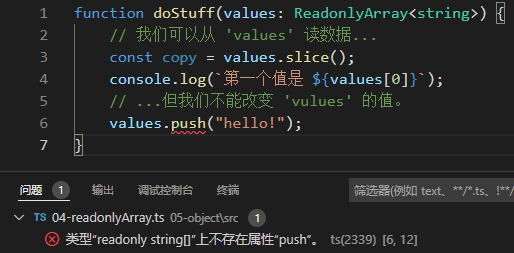``````new ReadonlyArray("red", "green", "blue");
``````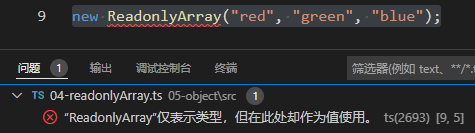``````const roArray: ReadonlyArray<string> = ["red", "green", "blue"];
``````

``````let x: readonly string[] = [];
let y: string[] = [];

x = y;
y = x;
``````## 6.11 元组类型

Tuple 类型是另一种 Array 类型，它确切地知道包含多少个元素，以及它在特定位置包含哪些类型。

``````type StringNumberPair = [string, number];
``````

``````function doSomething(pair: [string, number]) {
const a = pair;
const b = pair;
// ...
}
doSomething(["hello", 42])
``````

``````function doSomething(pair: [string, number]) {
const c = pair;
}
``````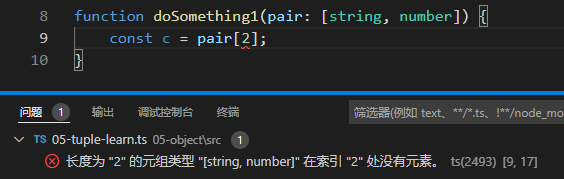``````function doSomething(stringHash: [string, number]) {
const [inputString, hash] = stringHash;
console.log(inputString);
console.log(hash);
}
``````

``````interface StringNumberPair {
// 专有属性
length: 2;
0: string;
1: number;
// 其他 'Array<string | number>' 成员...
slice(start?: number, end?: number): Array<string | number>;
}
``````

``````type Either2dOr3d = [number, number, number?];
function setCoordinate(coord: Either2dOr3d) {
const [x, y, z] = coord;
console.log(`提供的坐标有 \${coord.length} 个维度`);
}
``````

``````type StringNumberBooleans = [string, number, ...boolean[]];
type StringBooleansNumber = [string, ...boolean[], number];
type BooleansStringNumber = [...boolean[], string, number];
``````
• `StringNumberBooleans` 描述了一个元组，其前两个元素分别是字符串和数字，但后面可以有任意数量的布尔。
• `StringBooleansNumber` 描述了一个元组，其第一个元素是字符串，然后是任意数量的布尔运算，最后是一个数字。
• `BooleansStringNumber` 描述了一个元组，其起始元素是任意数量的布尔运算，最后是一个字符 串，然后是一个数字。

``````function readButtonInput(...args: [string, number, ...boolean[]]) {
const [name, version, ...input] = args;
console.log(name)
console.log(version)
console.log(input)

// ...
}

const data: boolean[] = [true, false, true]
const args:[string, number, ...boolean[]] = ["Hello world", 100, ...data]
console.log(args)
// [ 'Hello world', 100, true, false, true ]
// Hello world
// 100
// [ true, false, true ]
``````

``````function readButtonInput(name: string, version: number, ...input: boolean[]) {
// ...
}
``````

## 6.12 只读元组类型

``````function doSomething(pair: readonly [string, number]) {
// ...
}
``````

``````function doSomething(pair: readonly [string, number]) {
pair = "hello!";
}
``````

``````let point = [3, 4] as const;
function distanceFromOrigin([x, y]: [number, number]) {
return Math.sqrt(x ** 2 + y ** 2);
}
distanceFromOrigin(point);
``````

# TypeScript学习第七章: 类型操纵

## 7.0 从类型中创建类型

TS的类型系统非常强大, 因为它允许使用其他类型的术语来表达类型.

• 泛型型 – 带参数的类型
• Keyof 类型操作符- `keyof` 操作符创建新类型
• Typeof 类型操作符 – 使用 `typeof` 操作符来创建新的类型
• 索引访问类型 – 使用 `Type['a']` 语法来访问一个类型的子集
• 条件类型 – 在类型系统中像`if`语句一样行事的类型
• 映射类型 – 通过映射现有类型中的每个属性来创建类型
• 模板字面量类型 – 通过模板字面字符串改变属性的映射类型

## 7.1 泛型

### 7.1.1 Hello World

``````function echo(arg: number): number {
return arg
}
``````

``````function echo(arg: any): any {
return arg
}
``````

``````function echo<Type>(arg: Type): Type {
return arg
}
``````

``````let output = echo<string>("myString")
``````

``````let output = echo("myString")
``````

### 7.1.2 使用通用类型变量

``````function echo<Type>(arg: Type): Type {
return arg
}
``````

``````function loggingIdentity<Type>(arg: Type): Type {
console.log(arg.length);
return arg;
}
``````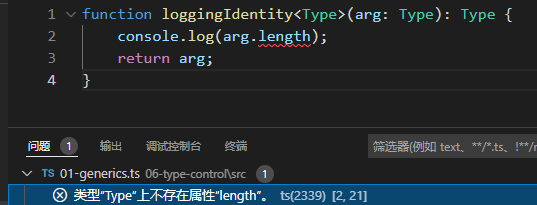``````function loggingIdentity<Type>(arg: Type[]): Type[] {
console.log(arg.length);
return arg;
}
``````

``````function loggingIdentity<Type>(arg: Array<Type>): Array<Type> {
console.log(arg.length); // 数组有一个.length，所以不会再出错了
return arg;
}
``````

### 7.1.3 泛型接口

``````function identity<Type>(arg: Type): Type {
return arg
}
let myIdentity: <Type>(arg: Type) => Type = identity
``````

``````function identity<Type>(arg: Type): Type {
return arg
}
let myIdentity: <Input>(arg: Input) => Input = identity
``````

``````function identity<Type>(arg: Type): Type {
return arg
}
let myIdentity: { <Type>(arg: Type): Type } = identity
``````

``````interface GenericIdentityFn {
<Type>(arg: Type): Type
}

function identity<Type>(arg: Type): Type {
return arg;
}
let myIdentity: GenericIdentityFn = identity
``````

``````interface GenericIdentityFn<Type> {
(arg: Type): Type;
}

function identity<Type>(arg: Type): Type {
return arg;
}
let myIdentity: GenericIdentityFn<number> = identity
``````

### 7.1.4 泛型类

``````class GenericNumber<NumType> {
zeroValue: NumType;
add: (x: NumType, y: NumType) => NumType
constructor(zeroValue:NumType, fn: (x: NumType, y: NumType) => NumType ) {
this.zeroValue = zeroValue
}
}

let myGenericNumber = new GenericNumber<number>(0, function (x, y) {
return x + y;
});

console.log(myGenericNumber.zeroValue) // 0
console.log(myGenericNumber.add(100, 200)) // 300
``````

### 7.1.5 泛型约束

``````function loggingIdentity<Type>(arg: Type): Type {
console.log(arg.length);
return arg;
}
``````

``````interface Lengthwise { //接口声明了一个具有number的对象
length: number;
}
function loggingIdentity<Type extends Lengthwise>(arg: Type): Type {
console.log(arg.length); // 现在我们知道它有一个 .length 属性，所以不再有错误了
return arg;
}
``````

``````loggingIdentity(3)
``````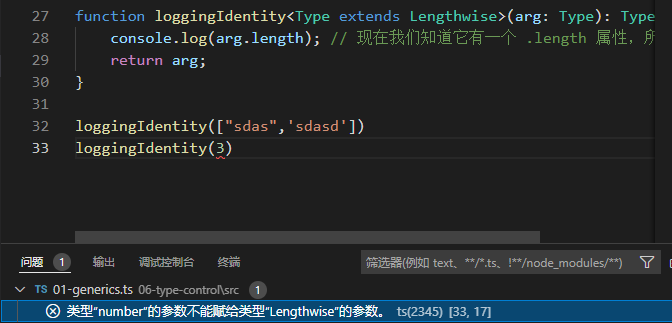``````loggingIdentity({ length: 10, value: 3 });
loggingIdentity(["sdas",'sdasd'])
``````

### 7.1.6 在泛型约束中使用类型参数

``````function getProperty<Type, Key extends keyof Type>(obj: Type, key: Key) {
return obj[key];
}
let x = { a: 1, b: 2, c: 3, d: 4 };
getProperty(x, "a");
getProperty(x, "m");
``````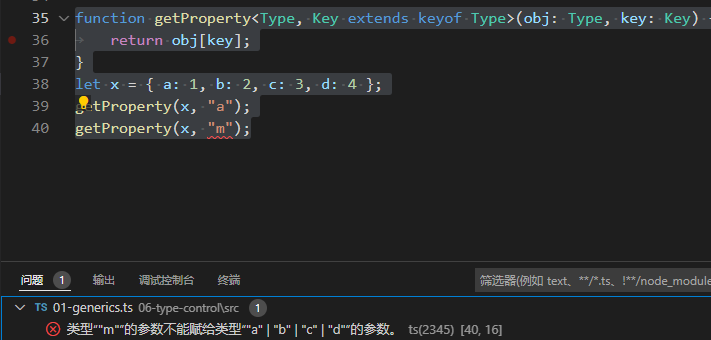### 7.1.7 在泛型中使用类类型

``````function create<Type>(c: { new (): Type }): Type {
return new c();
}
``````

``````class BeeKeeper {
hasMask: boolean = true;
}

class ZooKeeper {
nametag: string = "Mikle";
}

class Animal {
numLegs: number = 4;
}

class Bee extends Animal {
keeper: BeeKeeper = new BeeKeeper();
}
class Lion extends Animal {
keeper: ZooKeeper = new ZooKeeper();
}
function createInstance<A extends Animal>(c: new () => A): A {
return new c();
}

const lionNametag:string = createInstance(Lion).keeper.nametag;
console.log(lionNametag, BeeMask) // Mikle true
``````

## 7.2 `keyOf`类型操作符

`keyof` 运算符接收一个对象类型，并产生其键的字符串或数字字面联合。下面的类型`P``"x"|"y "`是同一类型。

``````type Point = { x: number; y: number };
type P = keyof Point;
const p1:P = 'x'
const p2:P = 'y'
``````

``````type Arrayish = { [n: number]: unknown };
type A = keyof Arrayish; // number
const a:A = 0

type Mapish = { [k: string]: boolean };
type M = keyof Mapish; // string|number
const m:M = 'a'
const m2:M = 10
``````

`keyof`类型在与映射类型结合时变得特别有用，我们将在后面进一步了解。

## 7.3 `typeof`类型操作符

JavaScript已经有一个 `typeof` 操作符，你可以在表达式上下文中使用。

``````// 输出 "string"
console.log(typeof "Hello world");
``````

TypeScript添加了一个 `typeof` 操作符，你可以在类型上下文中使用它来引用一个变量或属性的类型

``````let s = "hello";
let n: typeof s;
n = 'world'
n= 100
``````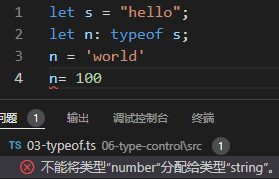``````type Predicate = (x: unknown) => boolean;
type K = ReturnType<Predicate>;
``````

``````function f() {
return { x: 10, y: 3 };
}
type P = ReturnType<f>
``````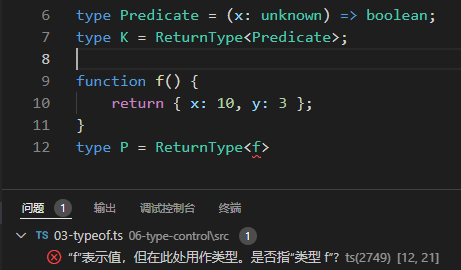``````function f() {
return { x: 10, y: 3 };
}
type P = ReturnType<typeof f>
``````

TypeScript 故意限制了你可以使用 `typeof` 的表达式种类。

``````// 我们认为使用 = ReturnType<typeof msgbox>
let shouldContinue: typeof msgbox("Are you sure you want to continue?");
``````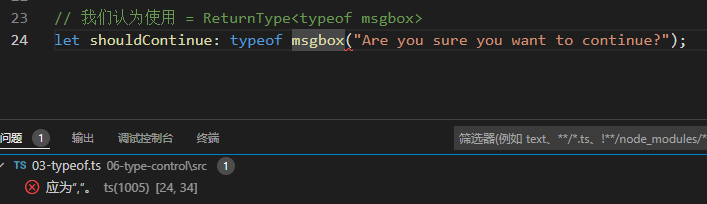## 7.4 索引访问类型

``````type Person = { age: number; name: string; alive: boolean };
type Age = Person["age"];
let age1: Age = 100
let age2: Age = "1100"
``````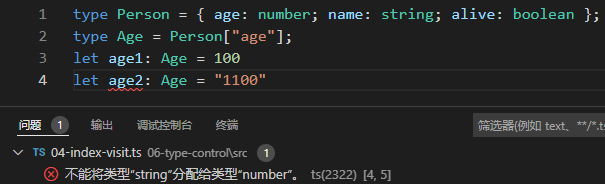``````interface Person {
name: string
age: number
alive: boolean
}

// type I1 = string | number
type I1 = Person["age" | "name"];
const i11:I1 = 100
const i12:I1 = ''

// type I2 = string | number | boolean
type I2 = Person[keyof Person];
const i21:I2 = ''
const i22:I2 = 100
const i23:I2 = false

// type I3 = Person[AliveOrName];
type AliveOrName = "alive" | "name";
const aon1:AliveOrName = 'alive'
const aon2:AliveOrName = 'name'
``````

``````type I1 = Person["alve"]
``````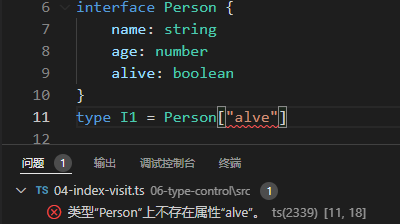``````const MyArray = [
{ name: "Alice", age: 15 },
{ name: "Bob", age: 23 },
{ name: "Eve", age: 38 },
];
/* type Person = {
name: string;
age: number;
} */
type Person = typeof MyArray[number];
const p:Person = {
name: 'xiaoqian',
age: 11
}
// type Age = number
type Age = typeof MyArray[number]["age"];
const age:Age = 11

// 或者
// type Age2 = number
type Age2 = Person["age"];
const age2:Age2 = 11
``````

``````const key = "age";
type Age = Person[key];
``````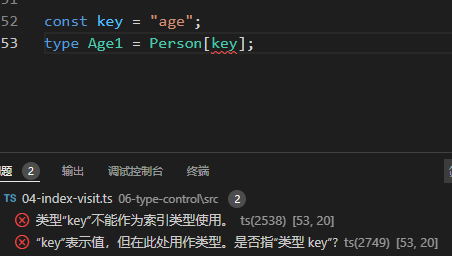``````type key = "age";
type Age = Person[key];
``````

## 7.5 条件类型

``````interface Animal {
live(): void;
}
interface Dog extends Animal {
woof(): void;
}
// type Example1 = number
type Example1 = Dog extends Animal ? number : string;
// type Example2 = string
type Example2 = RegExp extends Animal ? number : string;
``````

``````SomeType extends OtherType ? TrueType : FalseType;
``````

extends 左边的类型可以赋值给右边的类型时，那么你将得到第一个分支中的类型（”真 “分支）； 否则你将得到后一个分支中的类型（”假 “分支）

``````interface IdLabel {
id: number /* 一些字段 */;
}
interface NameLabel {
name: string /* 另一些字段 */;
}
function createLabel(id: number): IdLabel;
function createLabel(name: string): NameLabel;
function createLabel(nameOrId: string | number): IdLabel | NameLabel;
function createLabel(nameOrId: string | number): IdLabel | NameLabel {
throw "unimplemented";
}
``````

`createLabel`的这些重载描述了一个单一的JavaScript函数，该函数根据其输入的类型做出选择。注意 一些事情：

• 如果一个库必须在其API中反复做出同样的选择，这就会变得很麻烦。
• 我们必须创建三个重载：一个用于确定类型的情况（一个用于 `string` ，一个用于 `number` ），一个用于最一般的情况（取一个 `string | number` ）。对于 `createLabel` 所能处理的每一种新类型，重载的数量都会呈指数级增长。

``````type NameOrId<T extends number | string> = T extends number
? IdLabel
: NameLabel;
``````

``````interface IdLabel {
id: number /* some fields */;
}
interface NameLabel {
name: string /* other fields */;
}
type NameOrId<T extends number | string> = T extends number? IdLabel: NameLabel;

function createLabel<T extends number | string>(idOrName: T): NameOrId<T> {
throw "unimplemented";
}

// let a: NameLabel
let a = createLabel("typescript");

// let b: IdLabel
let b = createLabel(2.8);

// let c: NameLabel | IdLabel
let c = createLabel(Math.random() ? "hello" : 42);
``````

### 7.5.1 条件类型约束

``````type Message<T> = T["message"]
``````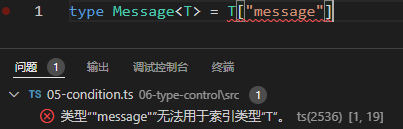``````type MessageOf<T extends { message: unknown }> = T["message"];
interface Email {
message: string;
}
type EmailMessageContents = MessageOf<Email>;
``````

``````type MessageOf<T> = T extends { message: unknown } ? T["message"] : never;

interface Email {
message: string;
}

interface Dog {
bark(): void;
}

// type EmailMessageContents = string
type EmailMessageContents = MessageOf<Email>;
const emc:EmailMessageContents = 'balabala...'
// type DogMessageContents = never
type DogMessageContents = MessageOf<Dog>;
const dmc:DogMessageContents = 'error' as never
``````

``````type Flatten<T> = T extends any[] ? T[number] : T;

// 提取出元素类型
// type Str = string
type Str = Flatten<string[]>

// 单独一个类型。
// type Num = number
type Num = Flatten<number>
``````

`Flatten` 被赋予一个数组类型时，它使用一个带有数字的索引访问来获取 `string[]` 的元素类型。 否则，它只是返回它被赋予的类型。

### 7.5.2 在条件类型内进行推理

``````type Flatten<Type> = Type extends Array<infer Item> ? Item : Type
``````

``````type GetReturnType<Type> = Type extends (...args: never[]) => infer Return? Return : never;

// type Num = number
type Num = GetReturnType<() => number>;
// type Str = string
type Str = GetReturnType<(x: string) => string>;
// type Bools = boolean[]
type Bools = GetReturnType<(a: boolean, b: boolean) => boolean[]>;
// 给泛型传入 string 类型，条件类型会返回 never
type Never = GetReturnType<string>
const nev:Never = 'error' as never
``````

``````declare function stringOrNum(x: string): number;
declare function stringOrNum(x: number): string;
declare function stringOrNum(x: string | number): string | number;

// type T1 = string | number
type T1 = ReturnType<typeof stringOrNum>;
``````

### 7.5.3 分布式条件类型

``````type ToArray<Type> = Type extends any? Type[] : never;
``````

``````type ToArray<Type> = Type extends any ? Type[] : never;
// type StrArrOrNumArr = string[] | number[]
type StrArrOrNumArr = ToArray<string | number>;
``````

``````string | number;
``````

``````ToArray<string> | ToArray<number>;
``````

``````string[] | number[];`
``````

``````type ToArrayNonDist<Type> = [Type] extends [any] ? Type[] : never;

// 'StrArrOrNumArr'不再是一个联合类型
// type StrArrOrNumArr = (string | number)[]
type StrArrOrNumArr = ToArrayNonDist<string | number>;
``````

## 7.6 映射类型

``````type OnlyBoolsAndHorses = {
[key: string]: boolean | Horse
}

const confroms: OnlyBoolsAndHorses = {
del: true,
rodney: false
}
``````

``````type OptionsFlags<Type> = {
[Property in keyof Type]: boolean;
};
``````

``````type FeatureFlags = {
darkMode: () => void;
newUserProfile: () => void;
};

/*
type FeatureOptions = {
darkMode: boolean;
newUserProfile: boolean;
}
*/
type FeatureOptions = OptionsFlags<FeatureFlags>;
``````

### 7.6.1 映射修改器

``````type CreateMutable<Type> = {
-readonly [Property in keyof Type]: Type[Property];
};

type LockedAccount = {
};

/*
type UnlockedAccount = {
id: string;
name: string;
}
*/

type UnlockedAccount = CreateMutable<LockedAccount>
``````
``````// 从一个类型的属性中删除 "可选" 属性
type Concrete<Type> = {
[Property in keyof Type]-?: Type[Property];
};
type MaybeUser = {
id: string;
name?: string;
age?: number;
};
/*
type User = {
id: string;
name: string;
age: number;
}
*/
type User = Concrete<MaybeUser>;
``````

### 7.6.2 通过 `as` 做`key`重映射

``````type MappedTypeWithNewProperties<Type> = {
[Properties in keyof Type as NewKeyType]: Type[Properties]
}
``````

`Capitalize<string & Property>`来是string首字母大写

``````type Getters<Type> = {
[Property in keyof Type as `get\${Capitalize<string & Property>}`]: () =>Type[Property]
};

interface Person {
name: string;
age: number;
location: string;
}
/*
type LazyPerson = {
getName: () => string;
getAge: () => number;
getLocation: () => string;
}
*/
type LazyPerson = Getters<Person>;
``````

`Exclude<Property, "kind">` 过滤掉key为”kind”的键

``````// 删除 "kind"属性
type RemoveKindField<Type> = {
[Property in keyof Type as Exclude<Property, "kind">]: Type[Property]
};

/*
type KindlessCircle = {
}
*/

interface Circle {
kind: "circle";
}

type KindlessCircle = RemoveKindField<Circle>;
``````

``````type EventConfig<Events extends { kind: string }> = {
[E in Events as E["kind"]]: (event: E) => void;
}

type SquareEvent = { kind: "square", x: number, y: number };
type CircleEvent = { kind: "circle", radius: number };
/*
type Config = {
square: (event: SquareEvent) => void;
circle: (event: CircleEvent) => void;
}
*/

type Config = EventConfig<SquareEvent | CircleEvent>
``````

### 7.6.3 进一步探索

``````type ExtractPII<Type> = {
[Property in keyof Type]: Type[Property] extends { pii: true } ? true : false;
};
/*
type ObjectsNeedingGDPRDeletion = {
id: false;
name: true;
}
*/
type DBFields = {
id: { format: "incrementing" };
name: { type: string; pii: true };
};
type ObjectsNeedingGDPRDeletion = ExtractPII<DBFields>
``````

# TypeScript学习第八章: 类

TS提供了对ES2015(ES6)中引入的`class`关键词的完全支持.

## 8.1 类成员

``````class Point {}
``````

### 8.1.1 类属性

``````class Point {
x: number
y: number
}

const pt = new Point()
pt.x = 0
pt.y = 0
``````

``````class Point {
x = 0
y = 0
}

const pt = new Point()
// Prints 0, 0
console.log(`\${pt.x}, \${pt.y}`)
``````

``````const pt = new Point();
pt.x = "0";
``````• `--strictPropertyInitialization`

`strictPropertyInitialization`设置控制是否需要在构造函数中初始化类字段。

``````class GoodGreeter {
name: string;
constructor() {
this.name = "hello";
}
}
``````

``````class OKGreeter {
// 没有初始化, 但没报错
name!: string
}
``````

### 8.1.2 `readonly`

``````class Greeter {
readonly name: string = "world";
constructor(otherName?: string) {
if (otherName !== undefined) {
this.name = otherName;
}
}
err() {
this.name = "not ok";
}
}

const g = new Greeter();
g.name = "also not ok";
``````### 8.1.3 构造器

``````class Point {
x: number
y: number

// 带默认值的正常签名
constructor(x = 0, y = 0) {
this.x = x;
this.y = y;
}
}
``````
``````class Point {
x: number;
y: string;

// 重载
constructor(x: number, y: string);
constructor(s: string);
constructor(xs: any, y?: any) {
if(y !== undefinded) {
this.x = xs
this.y = y
} else {
this.y = xs
}
}
}
``````

• 构造函数不能有类型参数–这属于外层类的声明，我们将在后面学习。
• 构造函数不能有返回类型注释——类的实例类型总是被返回的.

#### Super 调用

``````class Base {
k = 4;
}
class Derived extends Base {
constructor() {
// 在ES5中打印一个错误的值；在ES6中抛出异常。
console.log(this.k);
super();
}
}
``````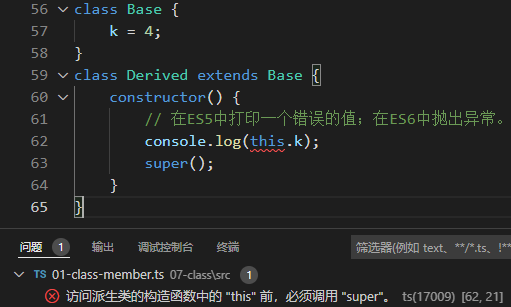### 8.1.4 方法

``````class Point {
x = 10;
y = 10;
scale(n: number): void {
this.x *= n;
this.y *= n;
}
}
``````

``````let x: number = 0;
class C {
x: string = "hello";
m() {
// 这是在试图修改第1行的'x'，而不是类属性。
x = "world";
}
}
``````

### 8.1.5 Getters/ Setters

``````class C {
_length = 0
get length() {
return this._length
}
set length(value) {
this._length = value
}
}
``````

TypeScript对访问器有一些特殊的推理规则:

• 如果存在 `get` ，但没有 `set` ，则该属性自动是只读的.

• 如果没有指定`setter`参数的类型，它将从`getter`的返回类型中推断出来.

• 访问器和设置器必须有相同的成员可见性.

``````class Thing {
_size = 0;

get size(): number {
return this._size;
}

set size(value: string | number | boolean) {
let num = Number(value);

// 不允许NaN、Infinity等
if (!Number.isFinite(num)) {
this._size = 0;
return;
}

this._size = num;
}
}
``````

### 8.1.6 索引签名

``````class MyClass {
[s: string]: boolean | ((s: string) => boolean);
check(s: string) {
return this[s] as boolean;
}
}
``````

## 8.2 类继承

### 8.2.1 `implements`子句

``````interface Pingable {
ping(): void;
}
class Sonar implements Pingable {
ping() {
console.log("ping!");
}
}
class Ball implements Pingable {
pong() {
console.log("pong!");
}
}
``````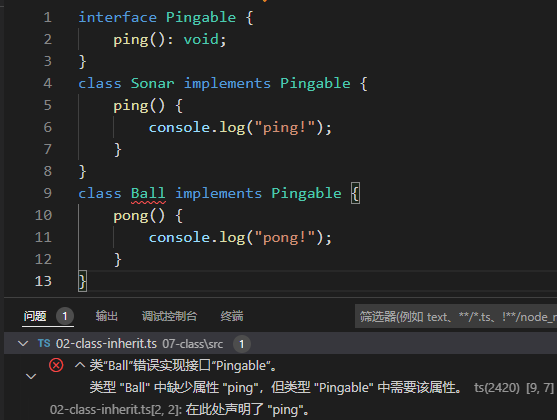#### 注意事项

``````interface Checkable {
check(name:string): boolean
}

class NameChecker implements Checkable {
check(s) {
// any: 注意这里没有错误
return s.toLowercse() === 'ok'
}
}
``````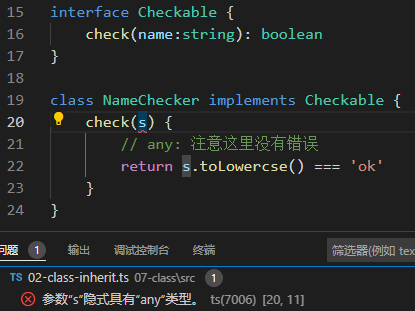``````interface A {
x: number;
y?: number;
}

class C implements A {
x = 0;
}

const c = new C();
c.y = 10;
``````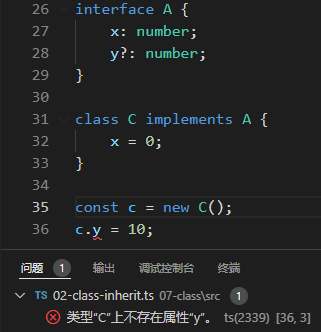### 8.2.2 `extends`子句

``````class Animal {
move() {
console.log("Moving along!");
}
}

class Dog extends Animal {
woof(times: number) {
for (let i = 0; i < times; i++) {
console.log("woof!");
}
}
}
const d = new Dog();

// 基类的类方法
d.move();

// 派生的类方法
d.woof(3);
``````

### 8.2.3 重写方法(遵守基类契约)

TypeScript强制要求派生类总是其基类的一个子类型.

``````class Base {
greet() {
console.log("Hello, world!");
}
}
class Derived extends Base {
greet(name?: string) {
if (name === undefined) {
super.greet();
} else {
console.log(`Hello, \${name.toUpperCase()}`);
}
}
}
const d = new Derived();
d.greet();
``````

``````// 通过基类引用对派生实例进行取别名
const b: Base = d;
// 没问题
b.greet();
``````

``````class Base {
greet() {
console.log("Hello, world!");
}
}
class Derived extends Base {
// 使这个参数成为必需的
greet(name: string) {
console.log(`Hello, \${name.toUpperCase()}`);
}
}
````````````const b: Base = new Derived();
// 崩溃，因为 "名称 "将是 undefined。
b.greet();
``````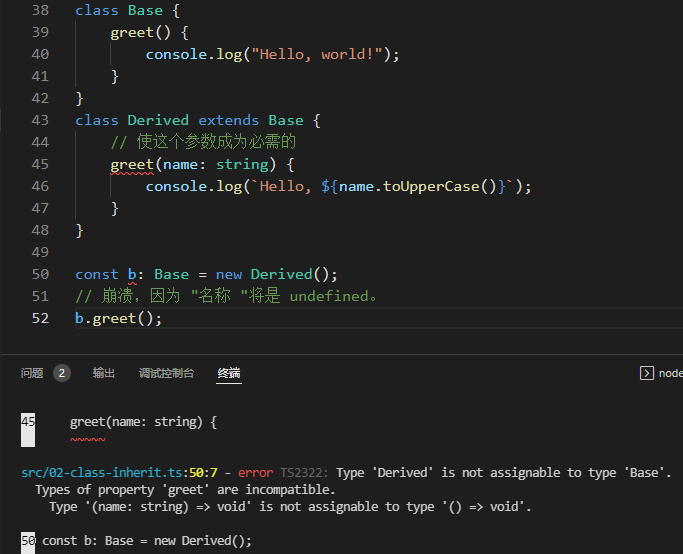### 8.2.4 初始化顺序

``````class Base {
name = "base";
constructor() {
console.log("My name is " + this.name);
}
}

class Derived extends Base {
name = "derived";
}
// 打印 "base", 而不是 "derived"
const d = new Derived();
``````

• 基类的字段被初始化
• 基类构造函数运行
• 派生类的字段被初始化
• 派生类构造函数运行

### 8.2.5 继承内置类型

``````class MsgError extends Error {

constructor(m: string) {
super(m);
}

sayHello() {
return "hello " + this.message;
}
}
``````

• 方法在构造这些子类所返回的对象上可能是未定义的，所以调用 `sayHello` 会导致错误。
• `instanceof`将在子类的实例和它们的实例之间被打破，所以 `(new MsgError()) instanceof MsgError` 将返回 `false`

``````class MsgError extends Error {
constructor(m: string) {
super(m);
// 明确地设置原型。
Object.setPrototypeOf(this, MsgError.prototype);
}
sayHello() {
return "hello " + this.message;
}
}
``````

## 8.3 成员的可见性

### 8.3.1 `public`

``````class Greeter {
public greet() {
console.log("hi!");
}
}

const g = new Greeter();
g.greet();
``````

### 8.3.2 `protected`

``````class Greeter {
public greet() {
console.log("Hello, " + this.getName());
}
protected getName() {
return "hi";
}
}

class SpecialGreeter extends Greeter {
public howdy() {
// 在此可以访问受保护的成员
console.log("Howdy, " + this.getName());
}
}
const g = new SpecialGreeter();
g.greet(); // 没有问题
g.getName(); // 无权访问
``````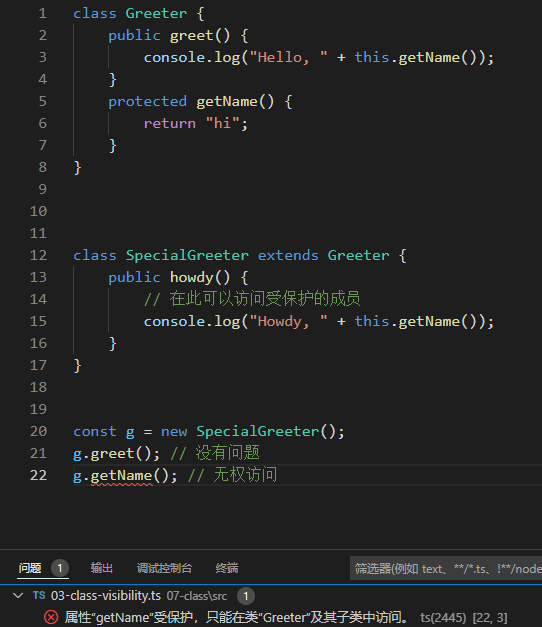• 受保护成员的暴露

``````class Base {
protected m = 10;
}

class Derived extends Base {
// 没有修饰符，所以默认为'公共'('public')
m = 15;
}

const d = new Derived();
console.log(d.m); // OK
``````

### 8.2.3 `private`

`private``protected`一样, 但不允许从子类中访问该成员.

``````class Base {
private x = 0;
}

const b = new Base();
// 不能从类外访问
console.log(b.x);
``````
``````class Base {
private x = 0;
}

const b = new Base();

class Derived extends Base {
showX() {
// 不能在子类中访问: 属性"x"为私有属性, 只能在类
console.log(this.x);
}
}
``````

• 跨实例的私有访问

TypeScript确实允许跨实例的私有访问:

``````class A {
private x = 10;

public sameAs(other: A) {
// 可以访问
return other.x === this.x;
}
}
``````
• 注意事项

``````class MySafe {
private secretKey = 12345;
}

// 在JS环境中...
const s = new MySafe();
// 将打印 12345
console.log(s.secretKey);
``````

`private` 也允许在类型检查时使用括号符号进行访问。这使得私有声明的字段可能更容易被单元测试之类的东西所访问，缺点是这些字段是软性私有的，不能严格执行私有特性。

``````class MySafe {
private secretKey = 12345;
}
const s = new MySafe();
// 在类型检查期间不允许
console.log(s.secretKey);
// 正确
console.log(s["secretKey"]);
``````

``````class Dog {
#barkAmount = 0;
personality = "happy";

constructor() {
// 0
console.log(this.#barkAmount)
}
}

const dog = new Dog()
// undefined
console.log(dog.barkAmount)
``````

``````"use strict";
var _Dog_barkAmount;

class Dog {
constructor() {
_Dog_barkAmount.set(this, 0);
this.personality = "happy";
}
}

_Dog_barkAmount = new WeakMap();
``````

## 8.4 静态成员

``````class MyClass {
static x = 0;
static printX() {
console.log(MyClass.x);
}
}
console.log(MyClass.x);
MyClass.printX();
``````

``````class MyClass {
private static x = 0;
}
console.log(MyClass.x);
``````

``````class Base {
static getGreeting() {
return "Hello world";
}
}

class Derived extends Base {
myGreeting = Derived.getGreeting();
}
``````

### 8.4.1 特殊静态名称

``````class S {
static name = "S!"
}
``````

### 8.4.2 为什么没有静态类？

TypeScript（和JavaScript）没有像C#和Java那样有一个叫做静态类的结构。

``````// 不需要 "static" class
class MyStaticClass {
static doSomething() {}
}

// 首选 (备选 1)
function doSomething() {}

// 首选 (备选 2)
const MyHelperObject = {
dosomething() {},
};
``````

## 8.5 类里的`static`区块

``````class Foo {
static #count = 0;

get count() {
return Foo.#count;
}

static {
try {
const lastInstances = {
length: 100
};
Foo.#count += lastInstances.length;
}
catch {}
}
}
``````

## 8.6 泛型类

``````class Box<Type> {
contents: Type
constructor(value: Type) {
this.contents = value
}
}

// const b: Box<string>
const b = new Box("hello")
``````

• 静态成员中的类型参数

``````class Box<Type> {
// 静态成员不能引用类的类型参数。
static defaultValue: Type;
}

// Box<string>.defaultValue = 'hello'
// console.log(Box<number>.defaultValue)
``````

## 8.7 类运行时的`this`

``````class MyClass {
name = "MyClass";
getName() {
return this.name;
}
}
const c = new MyClass();

const obj = {
name: "obj",
getName: c.getName,
};

// 输出 "obj", 而不是 "MyClass"
console.log(obj.getName())
``````

### 1. 箭头函数

``````class MyClass {
name = "MyClass";
getName = () => {
return this.name;
};
}

const c = new MyClass();
const g = c.getName;
// 输出 "MyClass"
console.log(g());
``````

• this 值保证在运行时是正确的，即使是没有经过TypeScript检查的代码也是如此。
• 这将使用更多的内存，因为每个类实例将有它自己的副本，每个函数都是这样定义的。
• 你不能在派生类中使用 `super.getName` ，因为在原型链中没有入口可以获取基类方法。

### 2. `this`参数

``````// 带有 "this" 参数的 TypeScript 输入
function fn(this: SomeType, x: number) {
/* ... */
}
``````
``````// 编译后的JavaScript结果
function fn(x) {
/* ... */
}
``````

TypeScript检查调用带有 `this` 参数的函数，是否在正确的上下文中进行。我们可以不使用箭头函数，而是在方法定义中添加一个 `this` 参数，以静态地确保方法被正确调用.

``````class MyClass {
name = "MyClass";
getName(this: MyClass) {
return this.name;
}
}

const c = new MyClass();
// 正确
c.getName();

// 错误
const g = c.getName;
console.log(g());
``````

• JavaScript调用者仍然可能在不知不觉中错误地使用类方法
• 每个类定义只有一个函数被分配，而不是每个类实例一个函数
• 基类方法定义仍然可以通过 super 调用。

## 8.8 `this`类型

``````class Box {
contents: string = "";
// (method) Box.set(value: string): this
set(value: string) {
this.contents = value;
return this;
}
}
``````

``````class ClearableBox extends Box {
clear() {
this.contents = "";
}
}
const a = new ClearableBox();

// const b: ClearableBox
const b = a.set("hello");
console.log(b)
``````

``````class Box {
content: string = "";
sameAs(other: this) {
return other.content === this.content;
}
}
const box = new Box()
console.log(box.sameAs(box))
``````

``````class Box {
content: string = "";
sameAs(other: this) {
return other.content === this.content;
}
}
class DerivedBox extends Box {
otherContent: string = "?";
}
const base = new Box();
const derived = new DerivedBox();
derived.sameAs(base);  // 报错 类型 "Box" 中缺少属性 "otherContent"，但类型 "DerivedBox" 中需要该属性。
``````

## 8.9 基于类型守卫的`this`（？？？不太会）

``````class FileSystemObject {
isFile(): this is FileRep {
return this instanceof FileRep;
}

isDirectory(): this is Directory {
return this instanceof Directory;
}

isNetworked(): this is Networked & this {
return this.networked;
}

constructor(public path: string, private networked: boolean) {
}

}
class FileRep extends FileSystemObject {
constructor(path: string, public content: string) {
super(path, false);
}
}
class Directory extends FileSystemObject {
children: FileSystemObject[];
}
interface Networked {
host: string;
}
const fso: FileSystemObject = new FileRep("foo/bar.txt", "foo");
if (fso.isFile()) {
// const fso: FileRep
fso.content;
} else if (fso.isDirectory()) {
// const fso: Directory
fso.children;
} else if (fso.isNetworked()) {
// const fso: Networked & FileSystemObject
fso.host;
}
``````

``````class Box <T> {
value?: T;
hasValue(): this is { value: T} {
return this.value !== undefined;
}
}

const box = new Box();
box.value = "Gameboy";

// (property) Box<unknown>.value?: unknownbox.value;
if (box.hasValue()) {
// (property) value: unknown
box.value;
}
``````

## 8.10 参数属性，构造函数参数转类属性

TypeScript提供了特殊的语法，可以将构造函数参数变成具有相同名称和值的类属性。这些被称为参数属性，通过在构造函数参数前加上可见性修饰符 `public``private``protected``readonly` 中的一个来创建。由此产生的字段会得到这些修饰符.

``````class Params {
constructor(public readonly x: number, protected y: number, private z: number)
{
// No body necessary
}
}
const a = new Params(1, 2, 3);
// (property) Params.x: number
console.log(a.x);
console.log(a.z);
``````

## 8.11 类表达式

``````const someClass = class<Type> {
content: Type;
constructor(value: Type) {
this.content = value;
}
};
// const m: someClass<string>
const m = new someClass("Hello, world");
``````

## 8.12 抽象类和成员

TypeScript中的类、方法和字段可以是抽象的。

``````abstract class Base {
abstract getName(): string;
printName() {
console.log("Hello, " + this.getName());
}
}
const b = new Base();
``````

``````class Derived extends Base {
getName() {
return "world";
}
}
const d = new Derived();
d.printName();
``````
• 抽象构造签名

``````function greet(ctor: typeof Base) {
const instance = new ctor();
instance.printName();
}
``````

TypeScript正确地告诉你，你正试图实例化一个抽象类。毕竟，鉴于greet的定义，写这段代码是完全合 法的，它最终会构造一个抽象类.

``````// 槽糕
greet(Base);
``````

``````function greet(ctor: new() => Base) {
const instance = new ctor();
instance.printName();
}
greet(Derived);
greet(Base);
``````

## 8.13 类之间的关系

``````class Point1 {
x = 0;
y = 0;
}
class Point2 {
x = 0;
y = 0;
}
// 正确
const p: Point1 = new Point2()
``````

``````class Person {
name: string;
age: number;
}
class Employee {
name: string;
age: number;
salary: number;
}
// 正确
const p: Person = new Employee();
``````

``````class Empty {
}
function fn(x: Empty) {
// 不能用'x'做任何事
}

// 以下调用均可
!fn(window);
fn({});
fn(fn);
``````

# TypeScript学习第九章：模块

JavaScript有很长的历史，有不同的方式来处理模块化的代码。TypeScript从2012年开始出现，已经实现了对许多这些格式的支持，但随着时间的推移，社区和JavaScript规范已经趋向于一种名为ES模块 （或ES6模块）的格式。你可能知道它是 import/export 语法。

ES Modules在2015年被加入到JavaScript规范中，到2020年，在大多数网络浏览器和JavaScript运行时中都有广泛的支持

## 9.2 非模块

``````export {}
``````

## 9.3 TypeScript中的模块

• 语法：我想用什么语法来导入和导出东西？
• 模块解析：模块名称（或路径）和磁盘上的文件之间是什么关系？
• 模块输出目标：我编译出来的JavaScript模块应该是什么样子的？

### 9.3.1 ES模块语法

``````// @filename: hello.ts
export default function helloWorld() {
console.log("Hello, world!");
}
``````

``````import hello from "./hello.js";
hello();
``````

``````// @filename: maths.ts
export var pi = 3.14;
export let squareTwo = 1.41;
export const phi = 1.61;
export class RandomNumberGenerator {}
export function absolute(num: number) {
if (num < 0) return num * -1;
return num;
}
``````

``````import { pi, phi, absolute } from "./maths.js";
console.log(pi);

// const absPhi: number
const absPhi = absolute(phi);
``````

### 9.3.2 额外的导入语法

``````import { pi as π } from "./maths.js";
// (alias)
var π: number
// import π
console.log(π);
``````

``````// @filename: maths.ts
export const pi = 3.14;
export default class RandomNumberGenerator {}

// @filename: app.ts
import RNGen, { pi as π } from "./maths.js";

// (alias) class RNGen
// import RNGen
RNGen;

// (alias) const π: 3.14
// import π
console.log(π);
``````

``````// @filename: app.ts
import * as math from "./maths.js";

console.log(math.pi);
// const positivePhi: number
const positivePhi = math.absolute(math.phi);
``````

``````// @filename: app.ts
import "./maths.js";

console.log("3.14");
``````

### 9.3.3 TypeScript特定的ES模块语法

``````// @filename: animal.ts
export type Cat = { breed: string; yearOfBirth: number };
export interface Dog {
breeds: string[];
yearOfBirth: number;
}
// @filename: app.ts
import { Cat, Dog } from "./animal.js";
type Animals = Cat | Dog;
``````

TypeScript用两个概念扩展了 import 语法，用于声明一个类型的导入。

• `import type`

``````// @filename: animal.ts
export type Cat = { breed: string; yearOfBirth: number };
export type Dog = { breeds: string[]; yearOfBirth: number };
export const createCatName = () => "fluffy";

// @filename: valid.ts
import type { Cat, Dog } from "./animal.js";
export type Animals = Cat | Dog;

// @filename: app.ts
import type { createCatName } from "./animal.js";
const name = createCatName();
``````
• 内联类型导入

TypeScript 4.5还允许以type为前缀的单个导入，以表明导入的引用是一个类型：

``````// @filename: app.ts
import { createCatName, type Cat, type Dog } from "./animal.js";

export type Animals = Cat | Dog;
const name = createCatName();
``````

### 9.3.4 ES模块语法与CommonJS行为

TypeScript有ES Module语法，它直接与CommonJS和AMD的 `require` 相关联。使用ES Module的`import` 在大多数情况下与这些环境的 `require` 相同，但这种语法确保你在TypeScript文件中与CommonJS的输出有1对1的匹配：

``````import fs = require("fs")
const code = fs.readFileSync("hello.ts", "utf8")
``````

## 9.4 CommonJS 语法

CommonJS是npm上大多数模块的交付格式。即使你使用上面的ES模块语法进行编写，对CommonJS语法的工作方式有一个简单的了解也会帮助你更容易地进行调试。

### 9.4.1 导出

``````function absolute(num: number) {
if (num < 0) return num * -1;
return num;
}
module.exports = {
pi: 3.14,
squareTwo: 1.41,
phi: 1.61,
absolute,
};

``````

``````const maths = require("maths");
// pi: any
maths.pi;
``````

``````const { squareTwo } = require("maths");
// const squareTwo: any
squareTwo;
``````

### 9.5 TypeScript的模块解析选项

TypeScript包括两种解析策略。经典和Node。当编译器选项 `module` 不是 `commonjs` 时，经典策略是默认的，是为了向后兼容。Node策略复制了Node.js在CommonJS模式下的工作方式，对 .ts 和 .d.ts 有额外的检查。

## 9.6 TypeScript的模块输出选项

• `target`, 它决定了哪些JS功能被降级（转换为在旧的JavaScript运行时运行），哪些保持不变
• `module`, 它决定了哪些代码用于模块之间的相互作用。

``````import { valueOfPi } from "./constants.js"
export const twoPi = valueOfPi * 2
``````
• ES2020
``````import { valueOfPi } from "./constants.js"
export const twoPi = valueOfPi * 2
``````
• CommonJS
``````"use strict";
Object.defineProperty(exports, "__esModule", { value: true });
exports.twoPi = void 0;
const constants_js_1 = require("./constants.js");
exports.twoPi = constants_js_1.valueOfPi * 2;
``````
• UMD
``````(function (factory) {
if (typeof module === "object" && typeof module.exports === "object") {
var v = factory(require, exports);
if (v !== undefined) module.exports = v;
}
else if (typeof define === "function" && define.amd) {
define(["require", "exports", "./constants.js"], factory);
}
})(function (require, exports) {
"use strict";
Object.defineProperty(exports, "__esModule", { value: true });
exports.twoPi = void 0;
const constants_js_1 = require("./constants.js");
exports.twoPi = constants_js_1.valueOfPi * 2;
});
``````

## 9.7 TypeScript 命名空间

TypeScript有自己的模块格式，称为 `命名空间(namespaces)` ，这比ES模块标准要早。这种语法对于创建复杂的定义文件有很多有用的功能，并且在 DefinitelyTyped中仍然被积极使用。虽然没有被废弃，但命名空间中的大部分功能都存在于ES Modules中，我们建议你使用它来与JavaScript的方向保持一致。 你可以在`namespaces参考页`中了解更多关于命名空间的信息。